# RS Aggarwal Solutions for Class 9 Chapter 2: Polynomials

In order to have a good academic score in Mathematics the important thing to be done by the students is to solve the questions of each and every exercise. RS Aggarwal Solutions for Class 9 is prepared by a team of experts who work with their full potential to help the students excel in their exams. Step by step solutions to each question in simple language understandable by the students is the primary aim of the experts.

The chapters in class 9 are of much importance as these topics can also be continued in further levels of education. The students can score good marks in the exams by solving all the questions and cross checking the answers with the RS Aggarwal Solutions prepared by our faculties. RS Aggarwal Solutions for Class 9 Chapter 2 Polynomials are provided here.

## RS Aggarwal Solutions for Class 9 Chapter 2: Polynomials Download PDF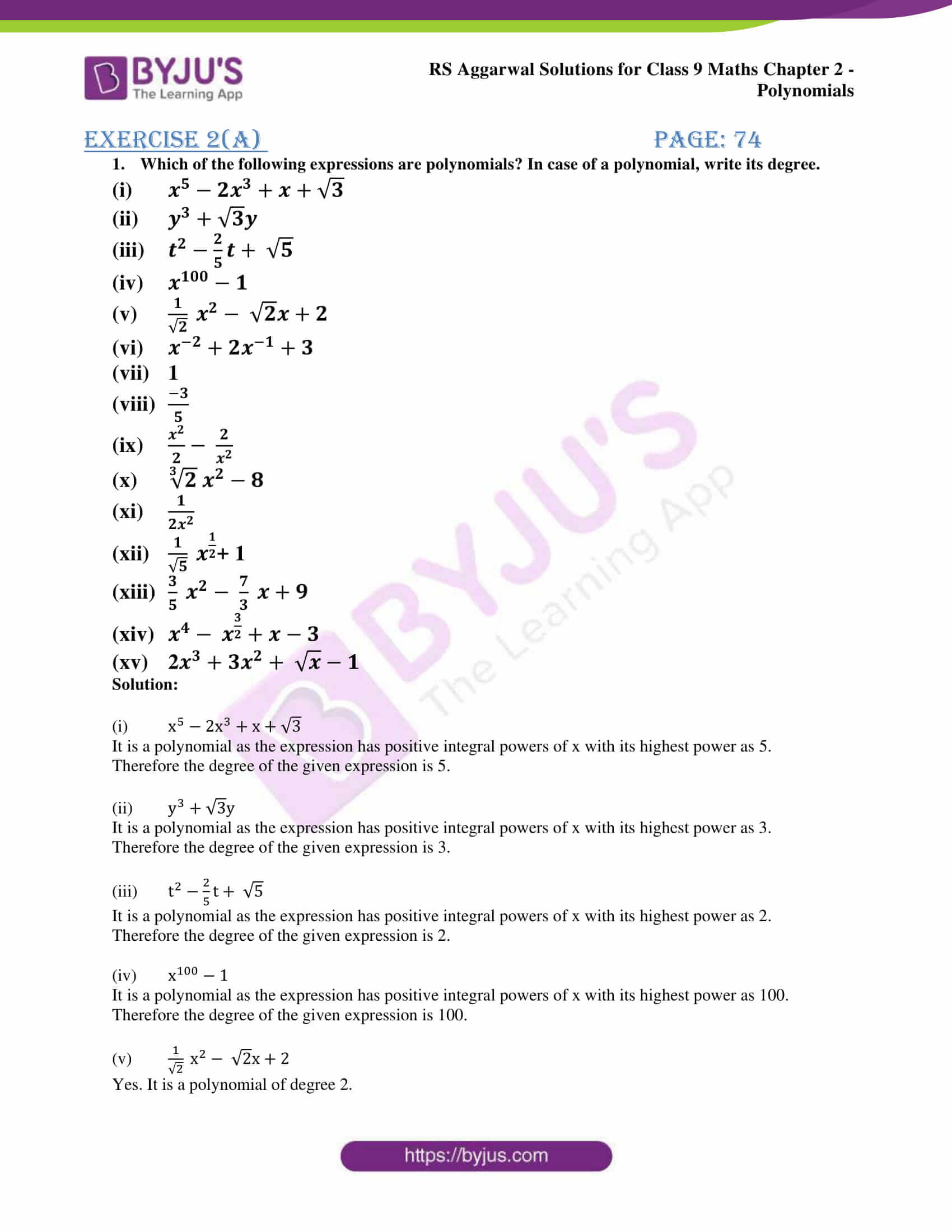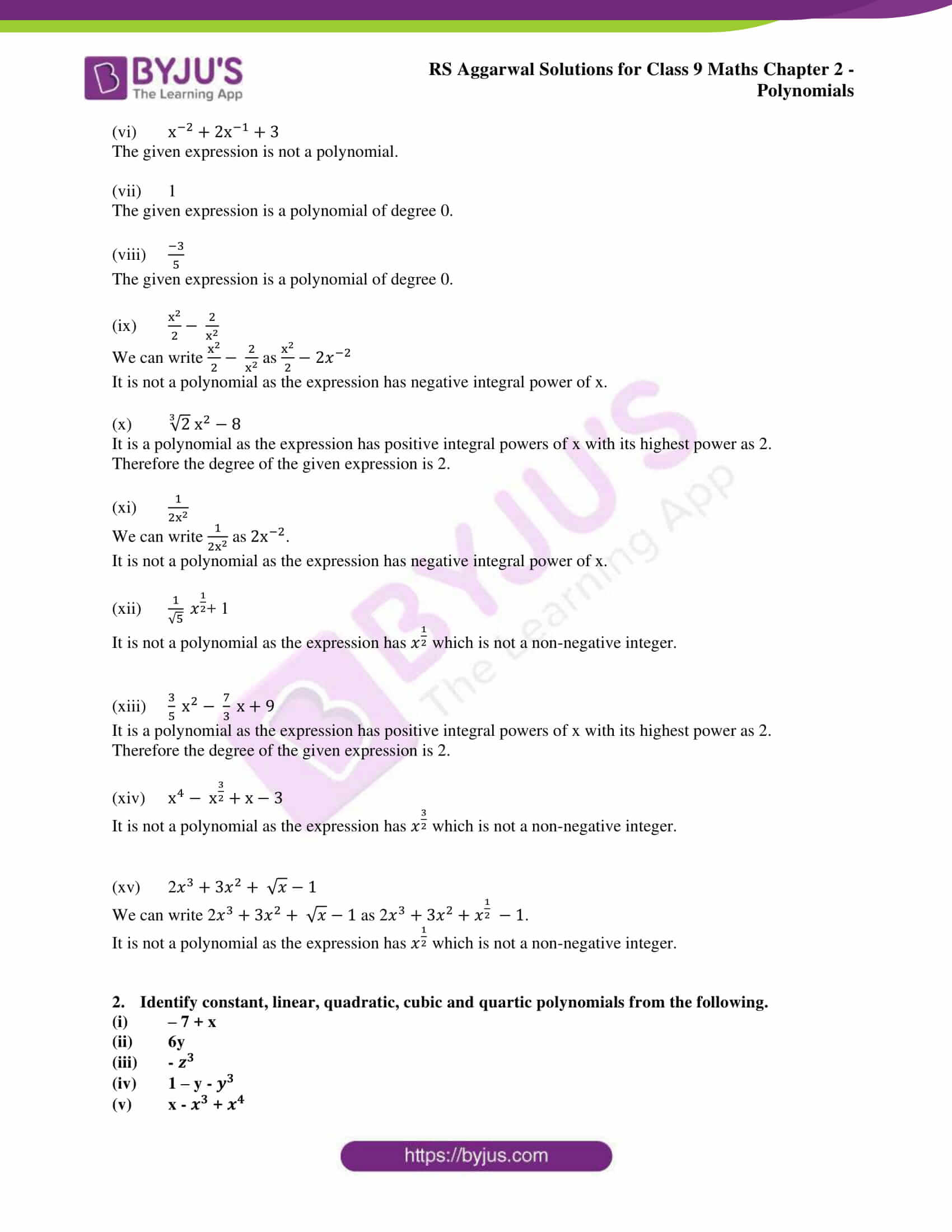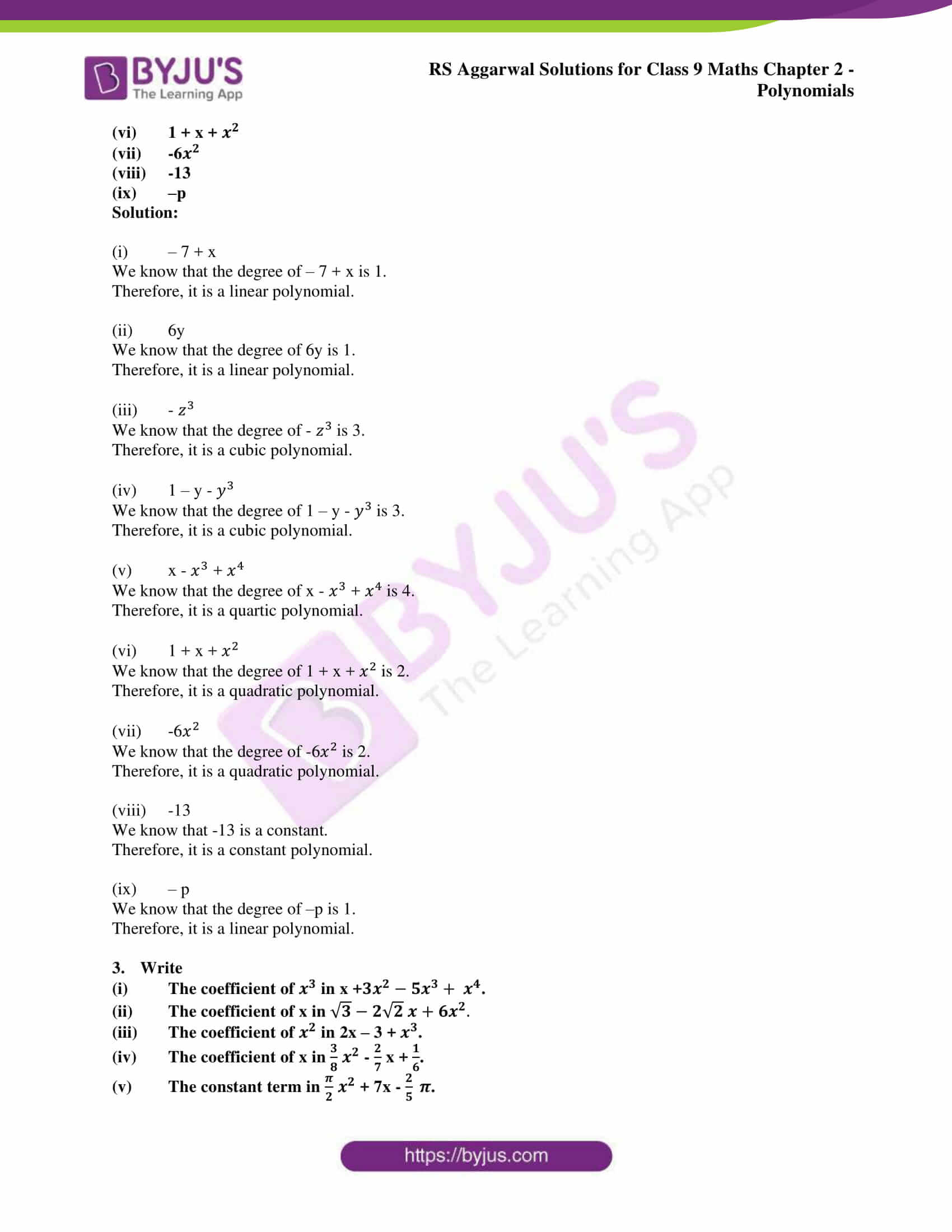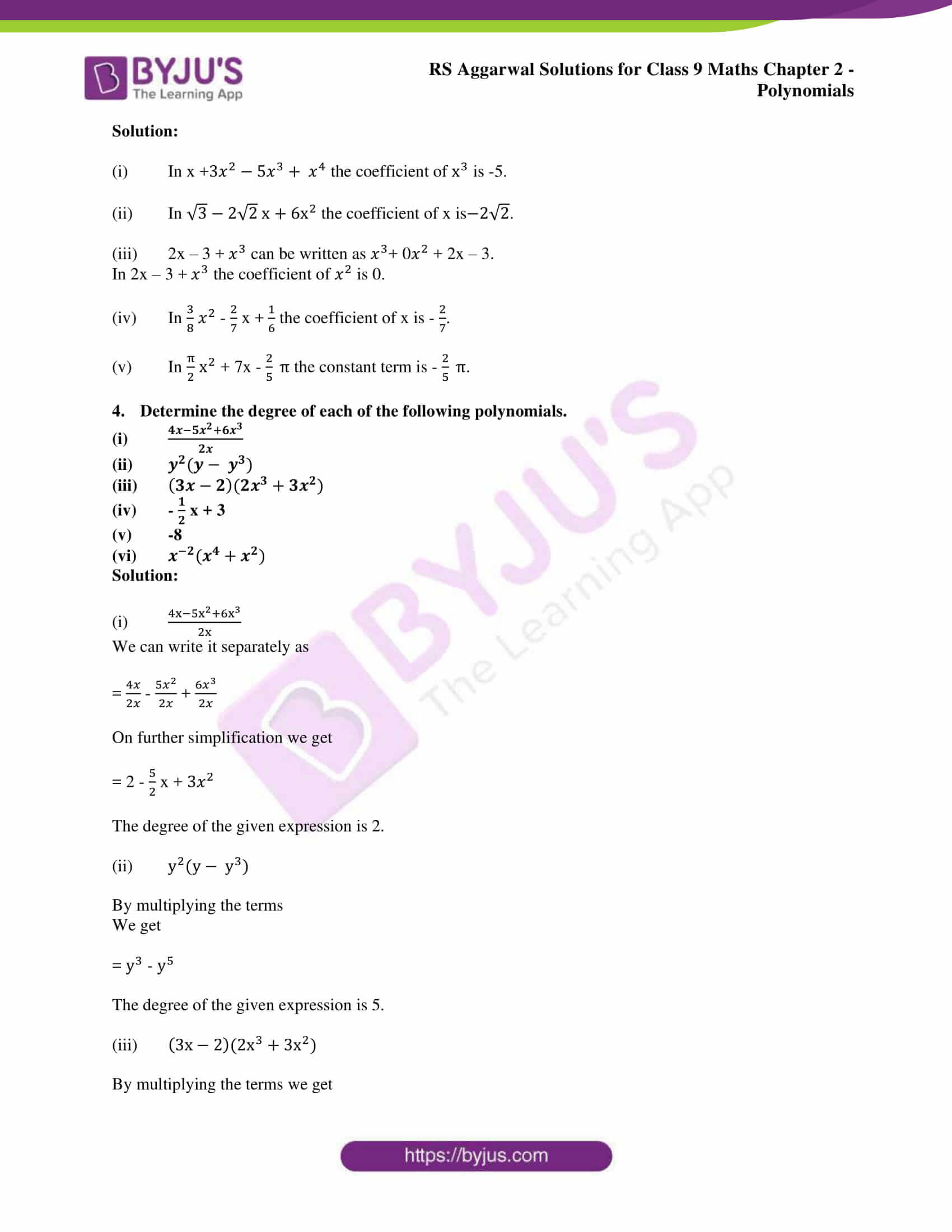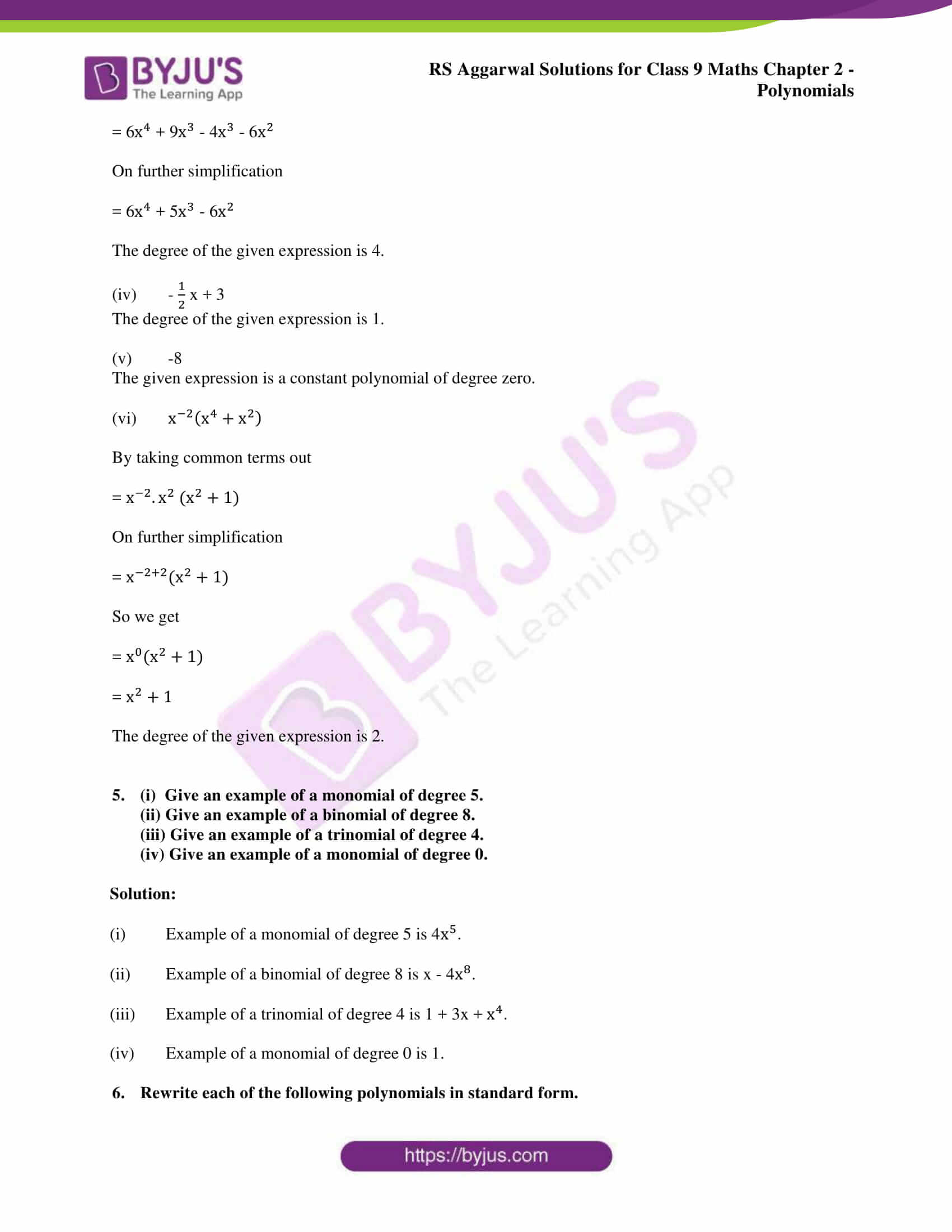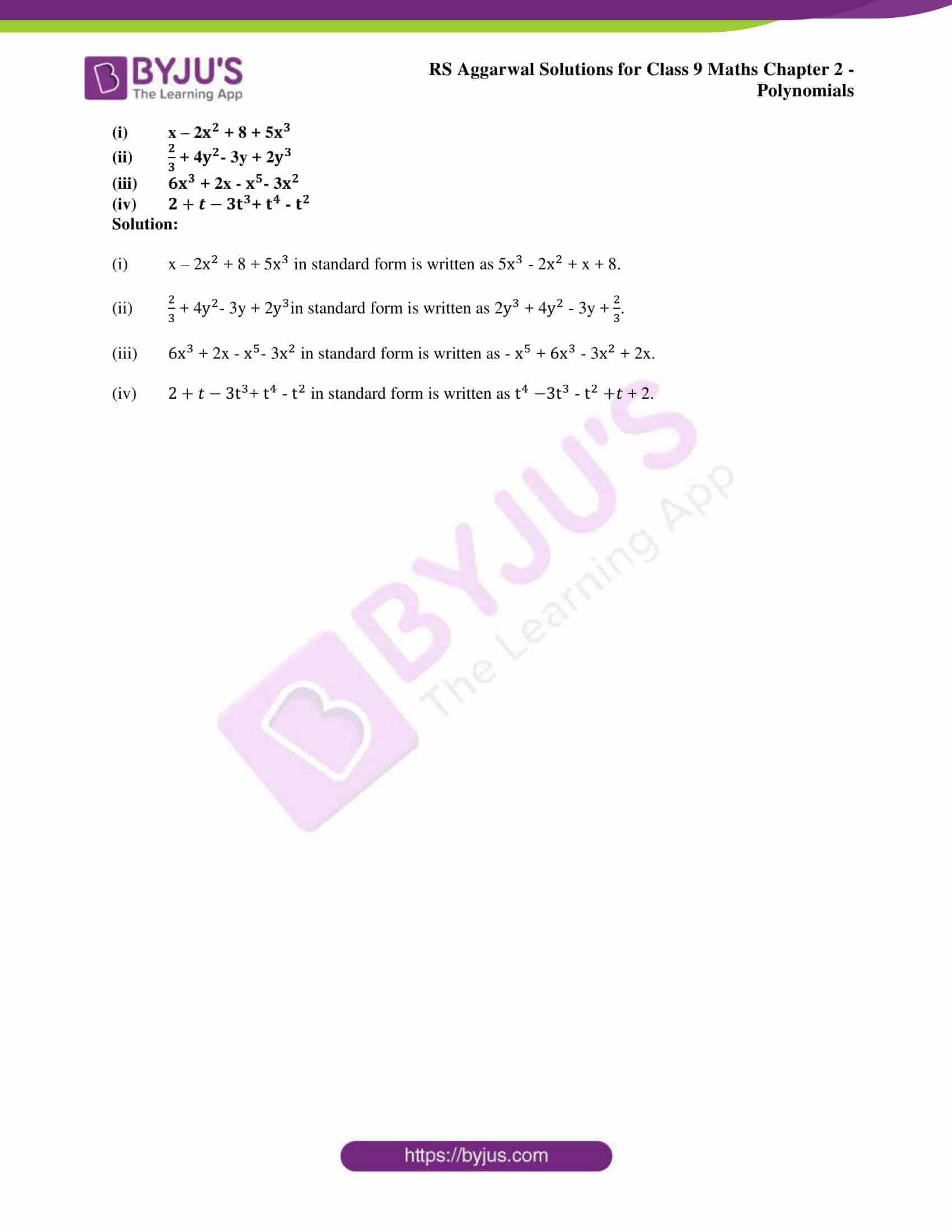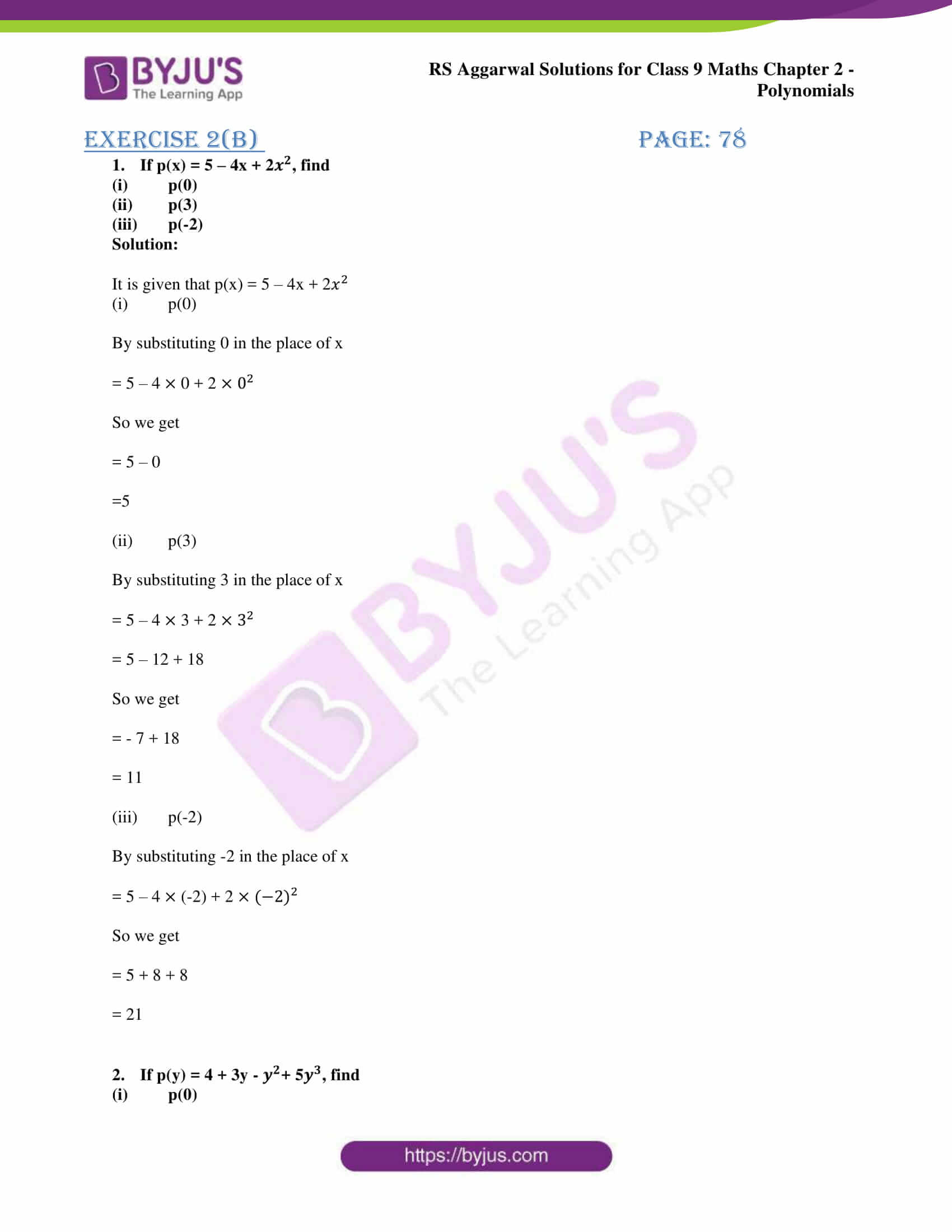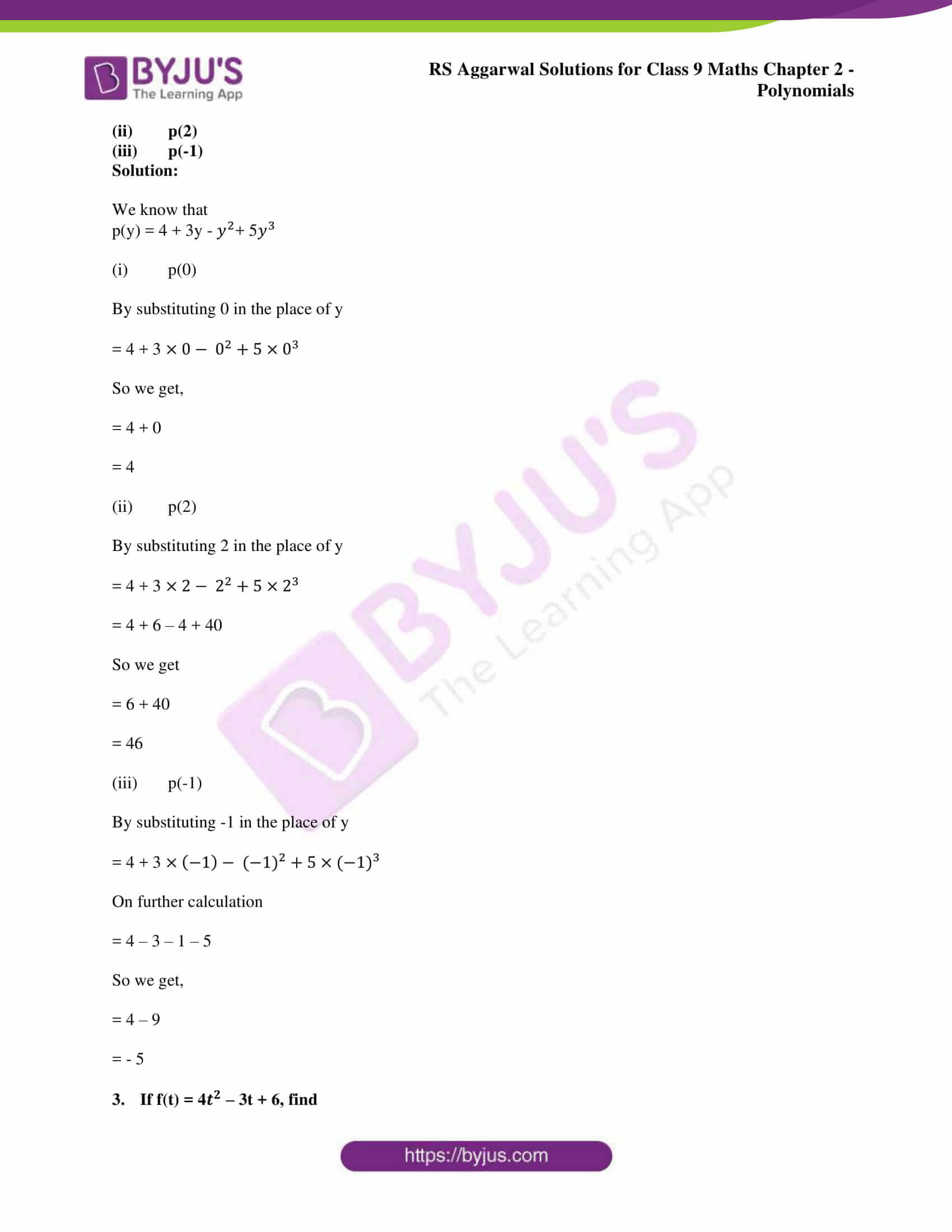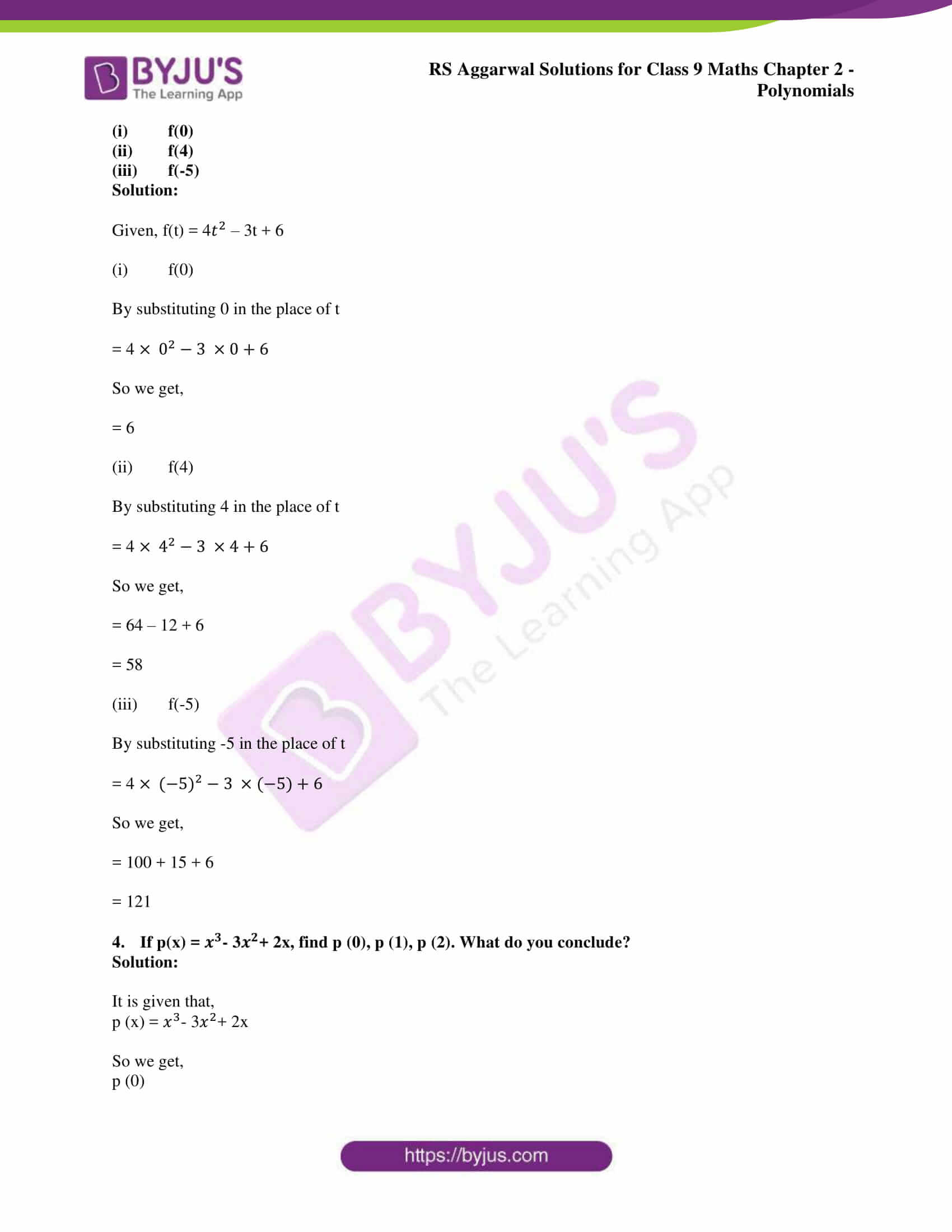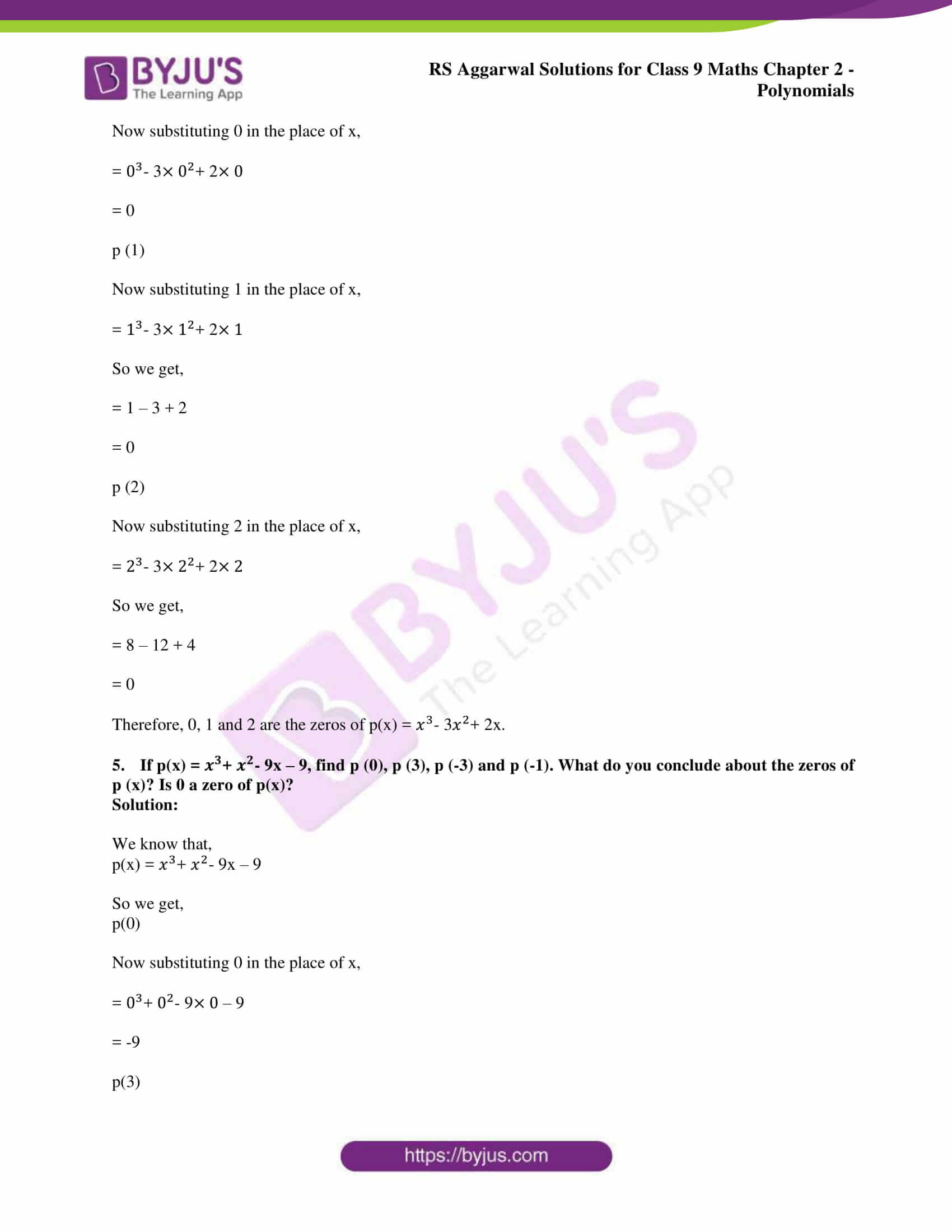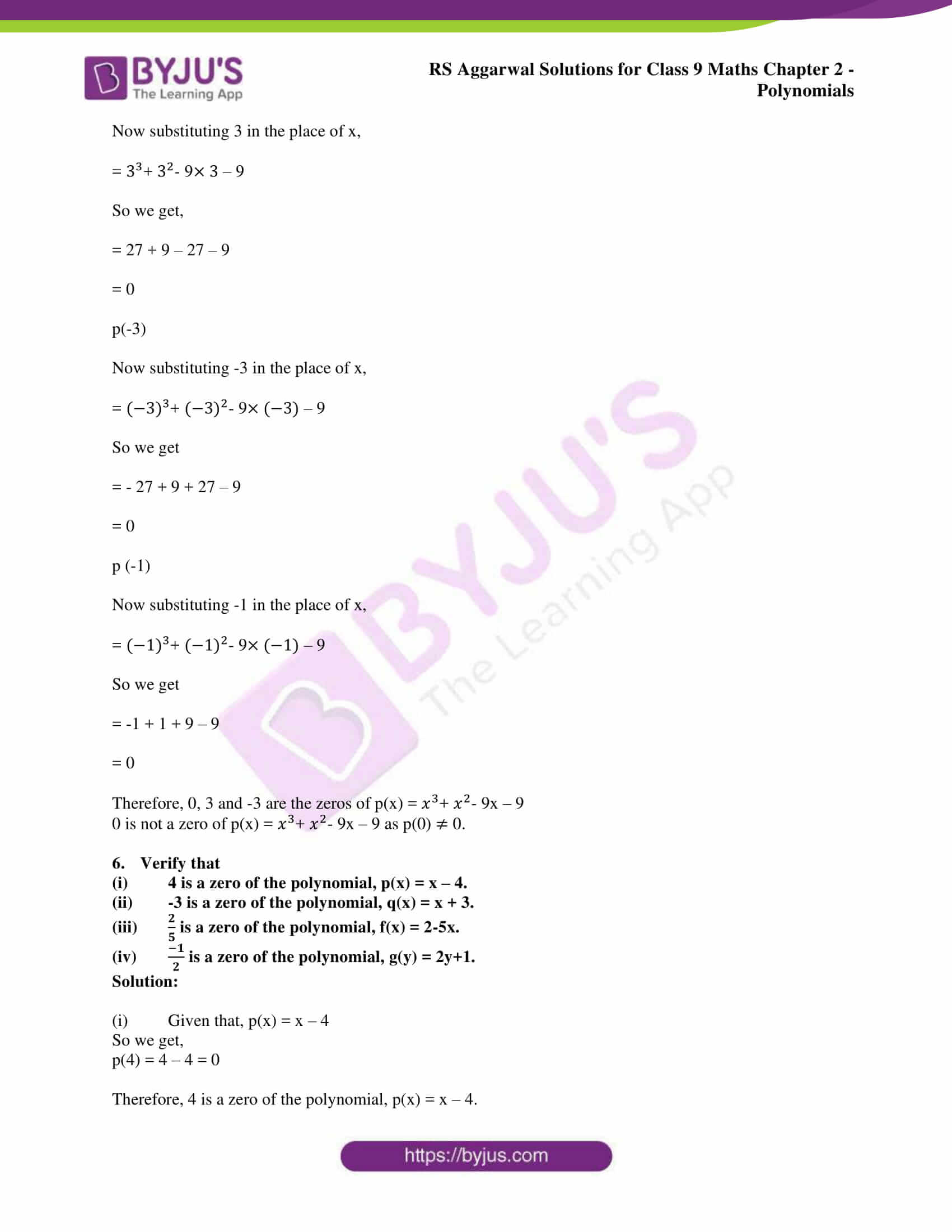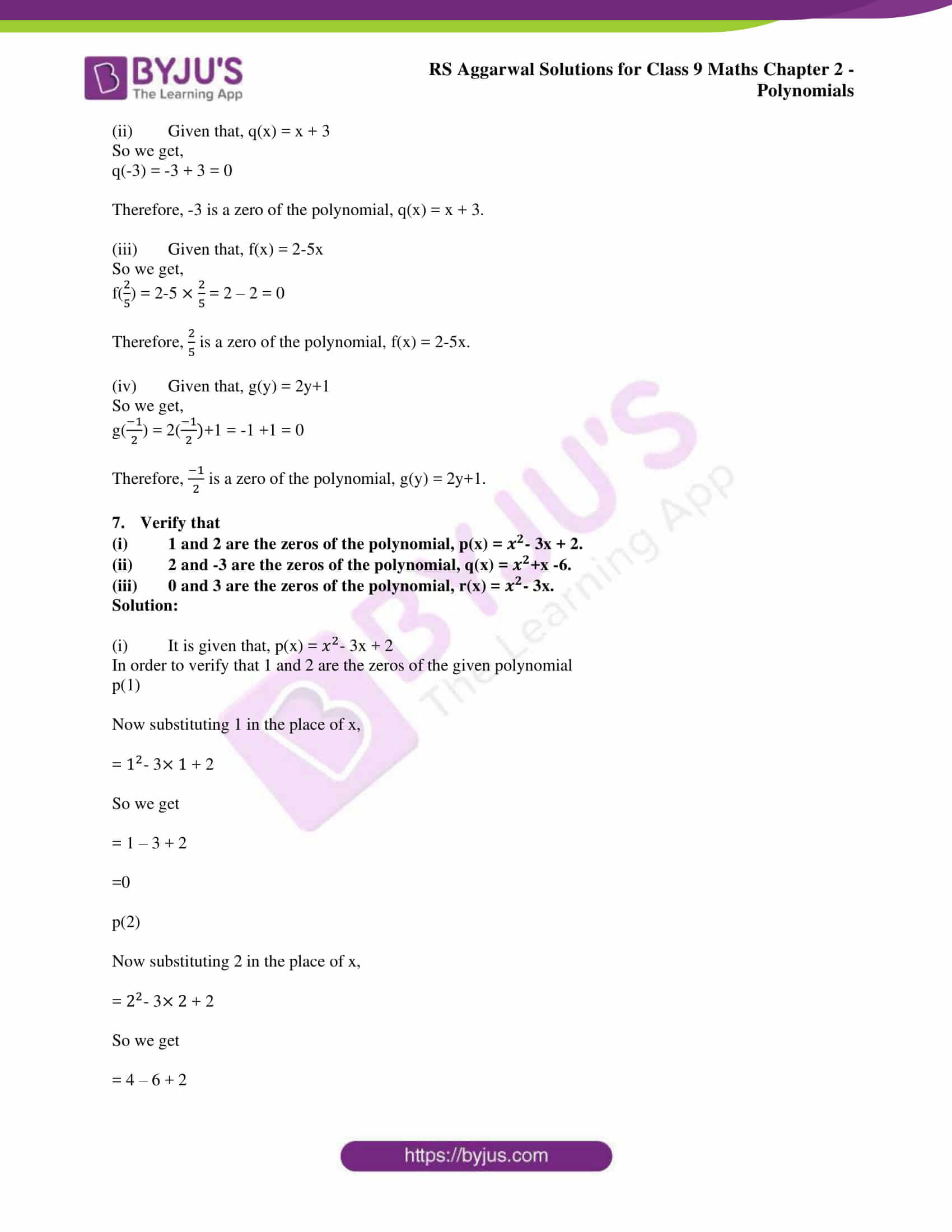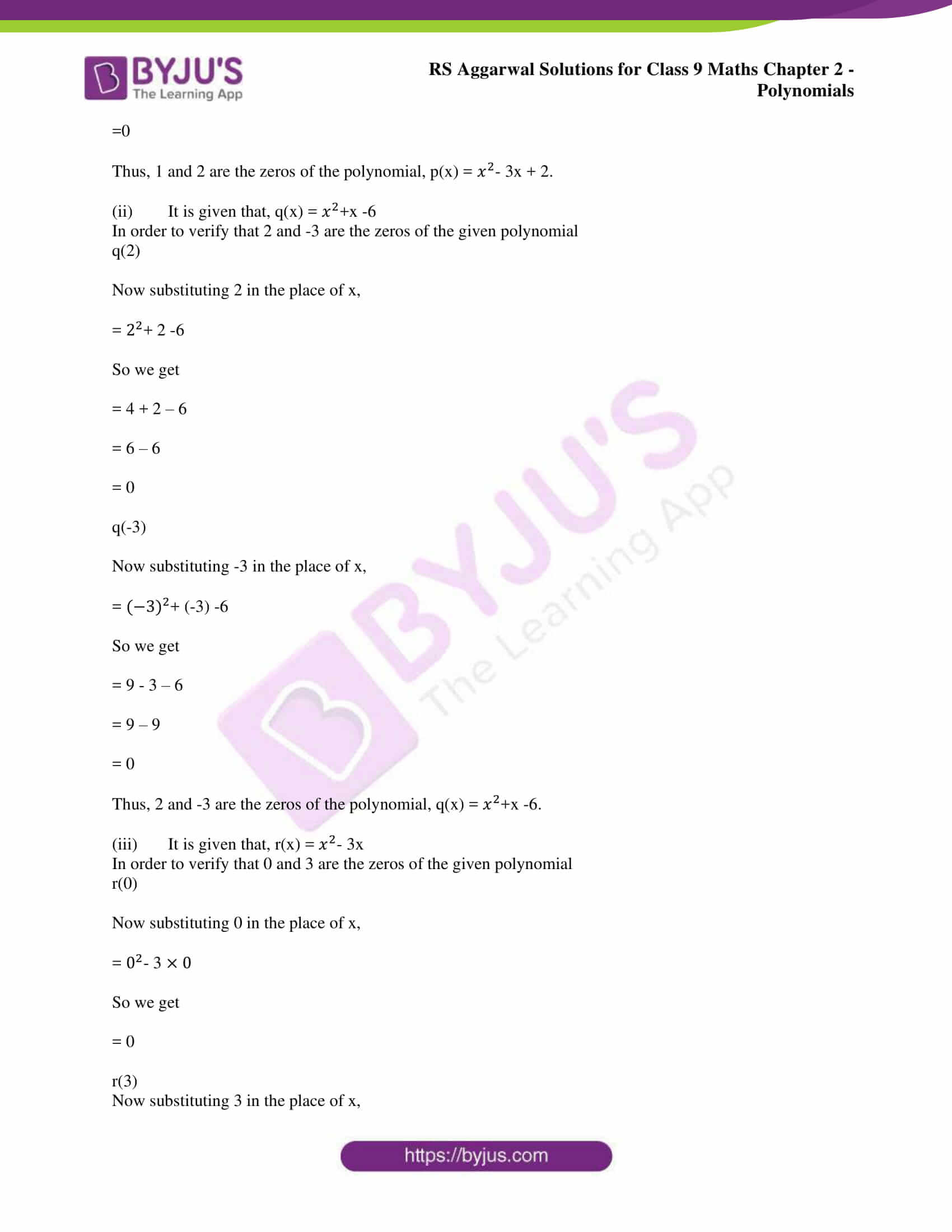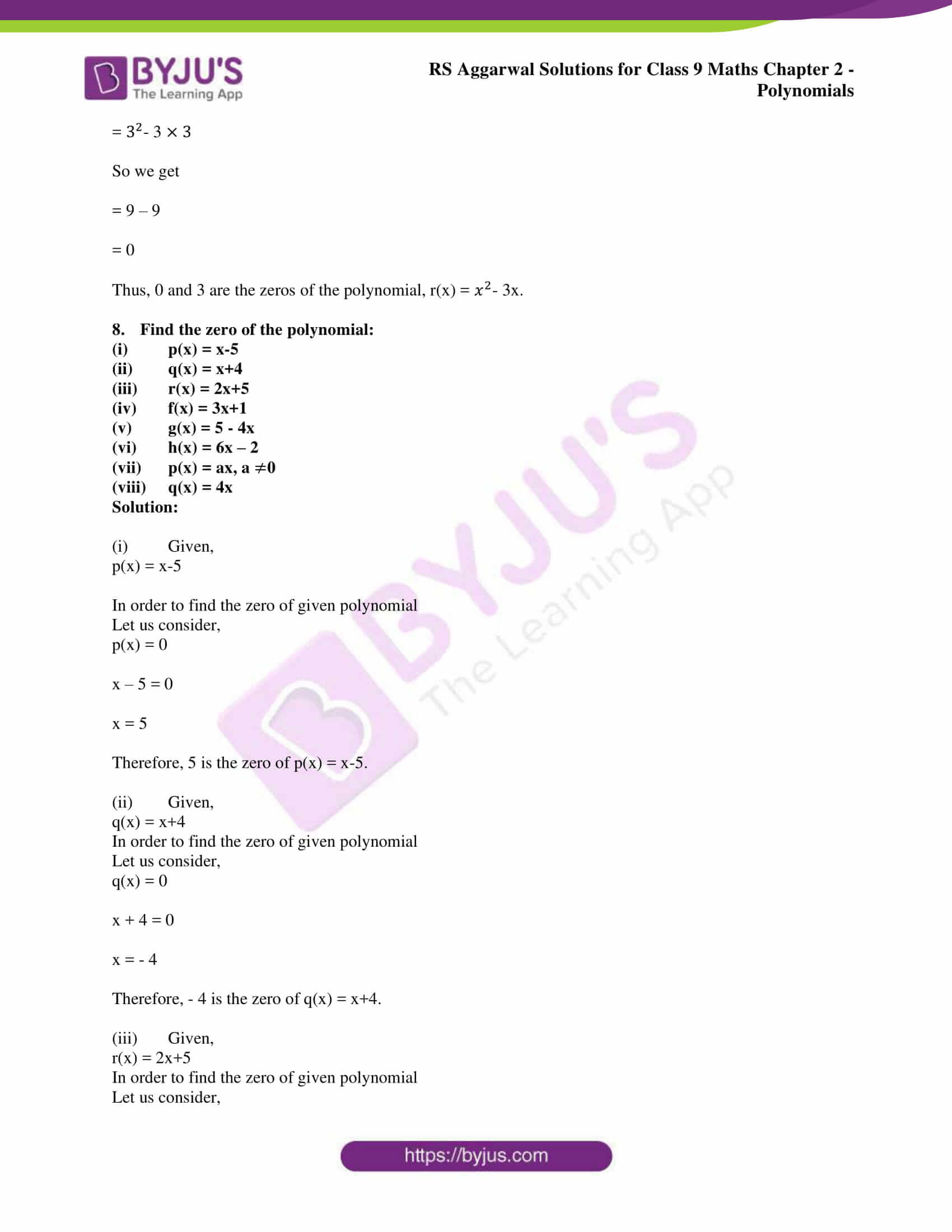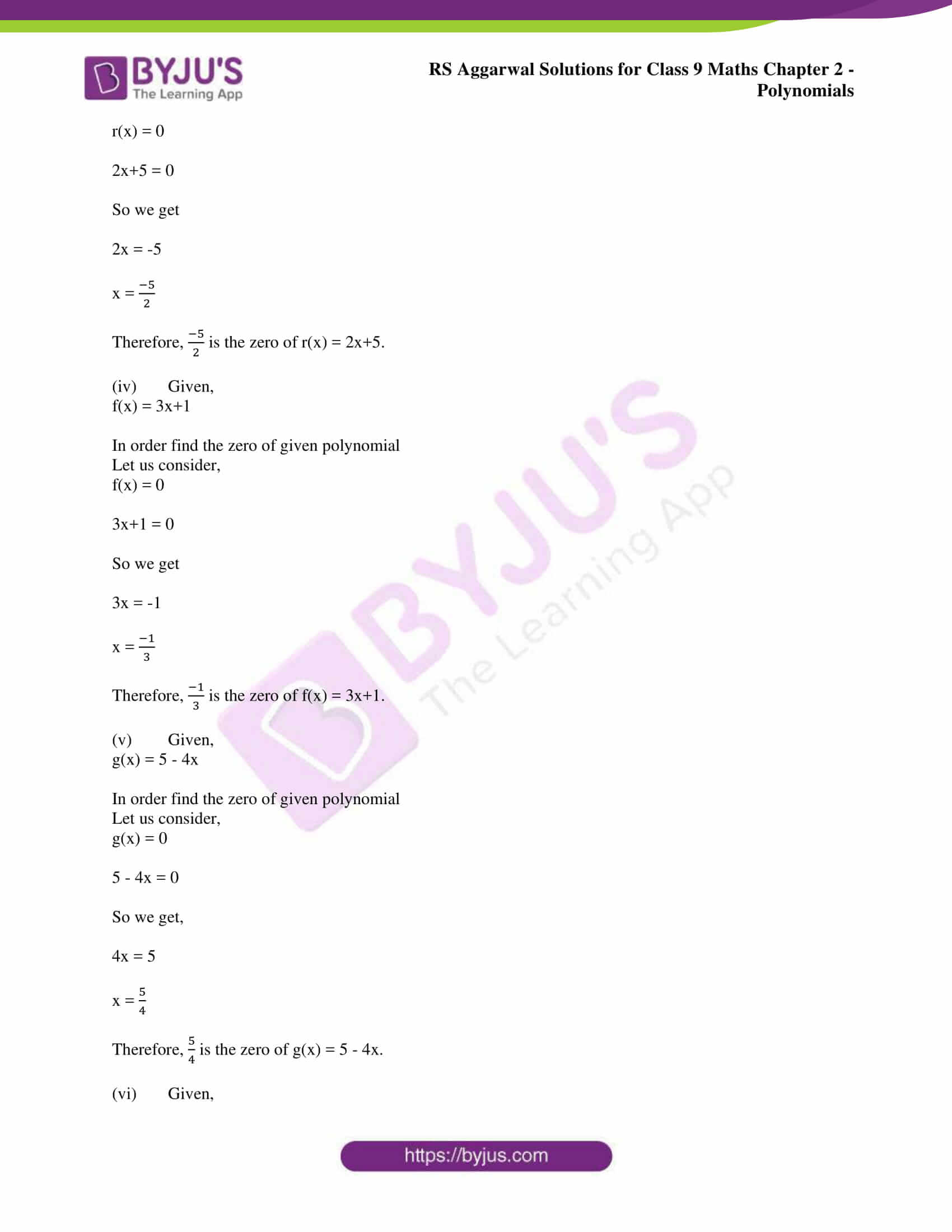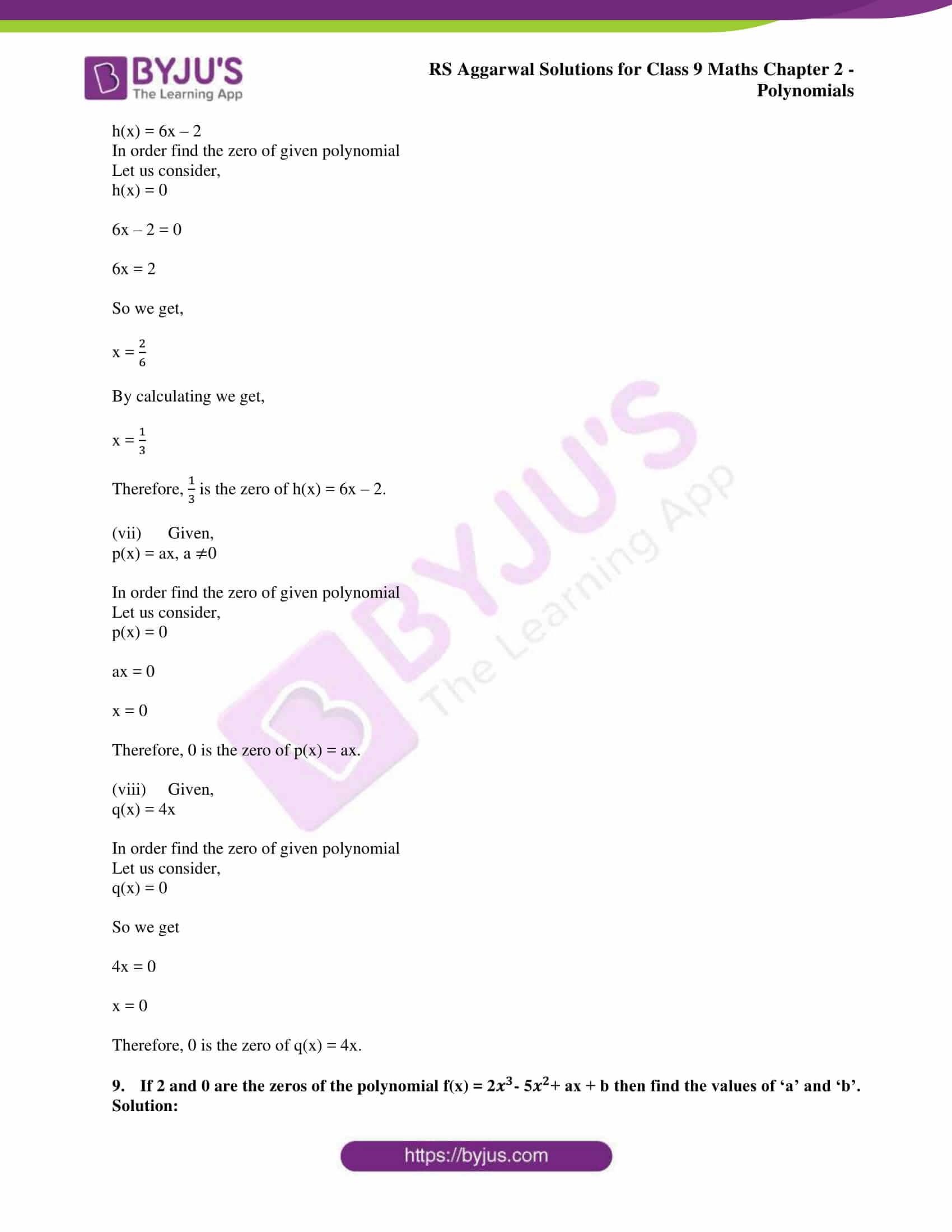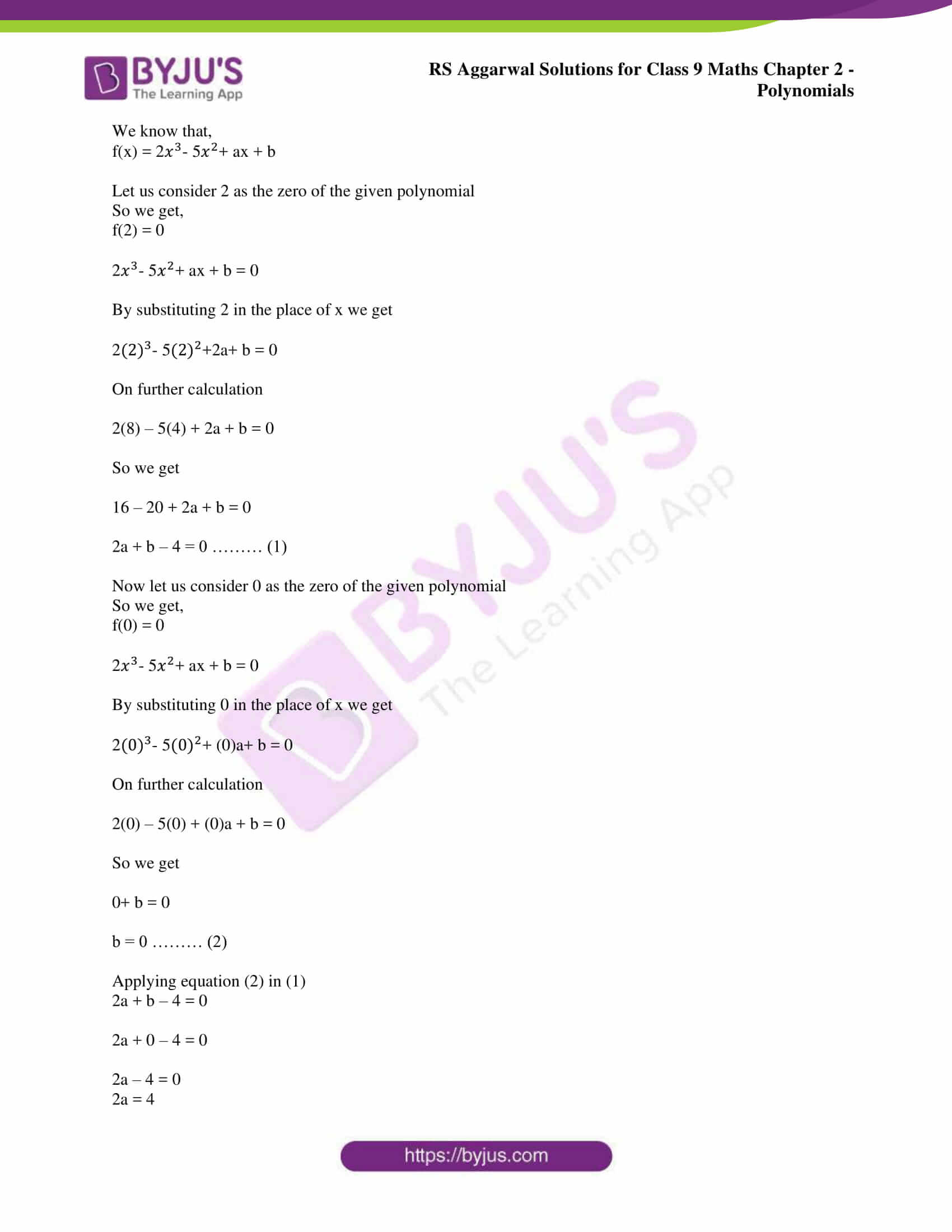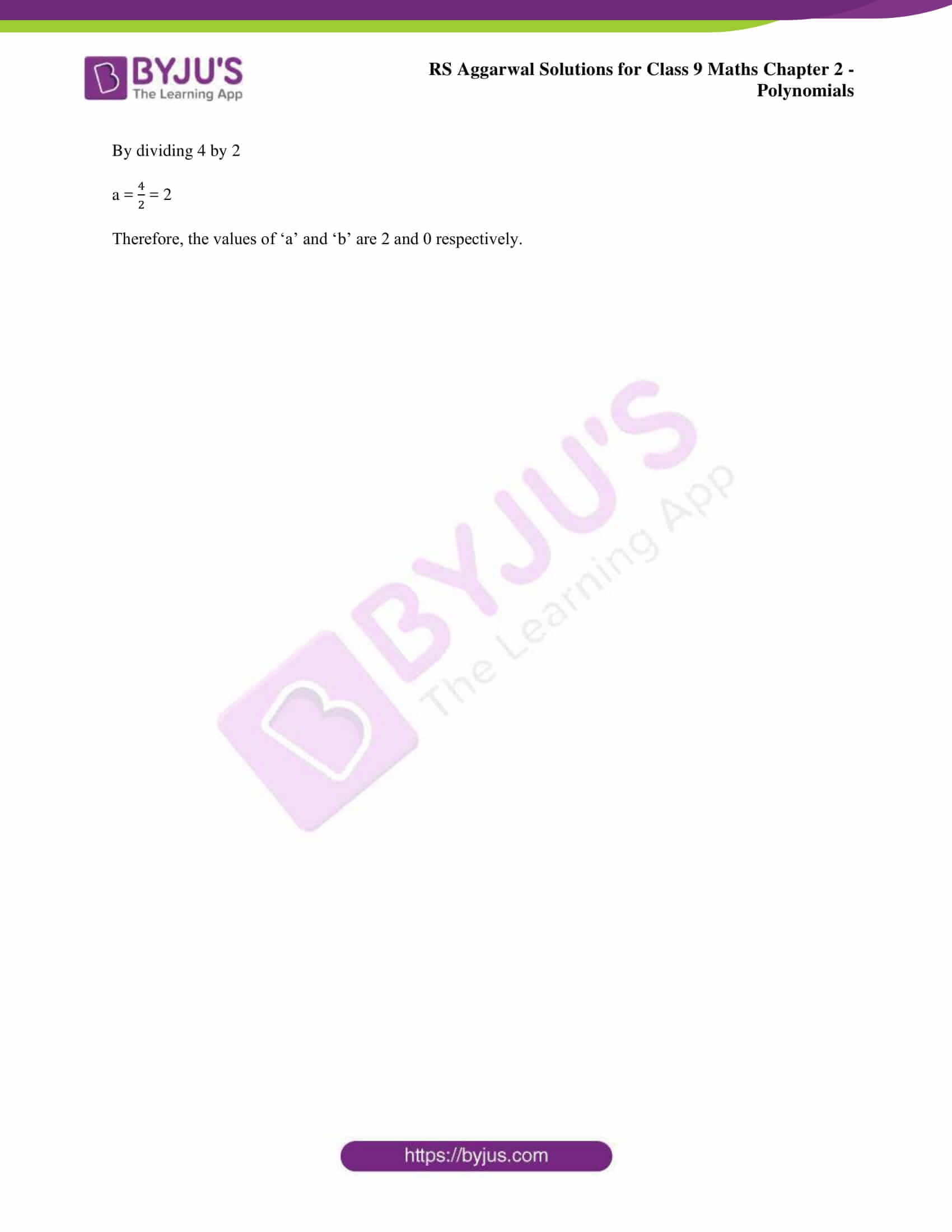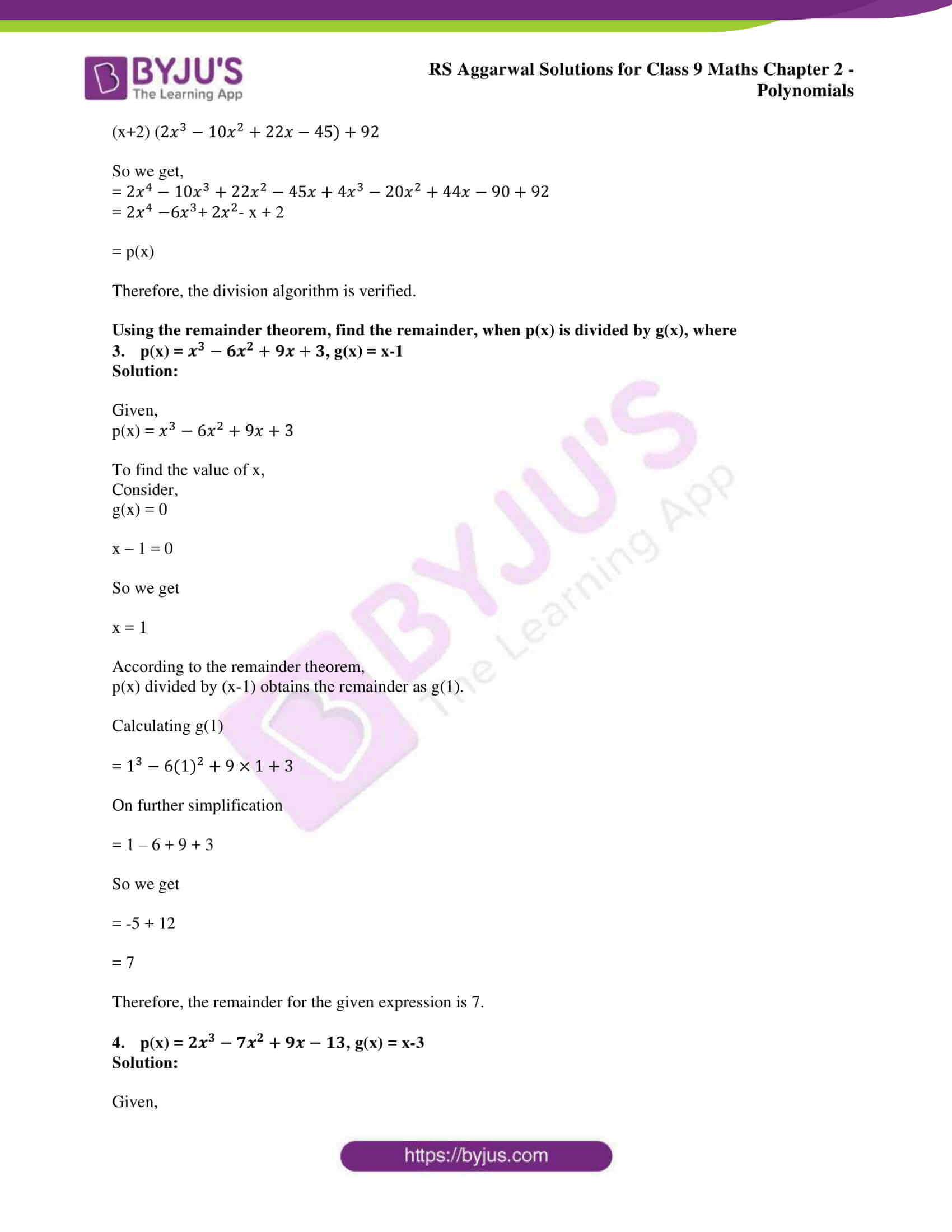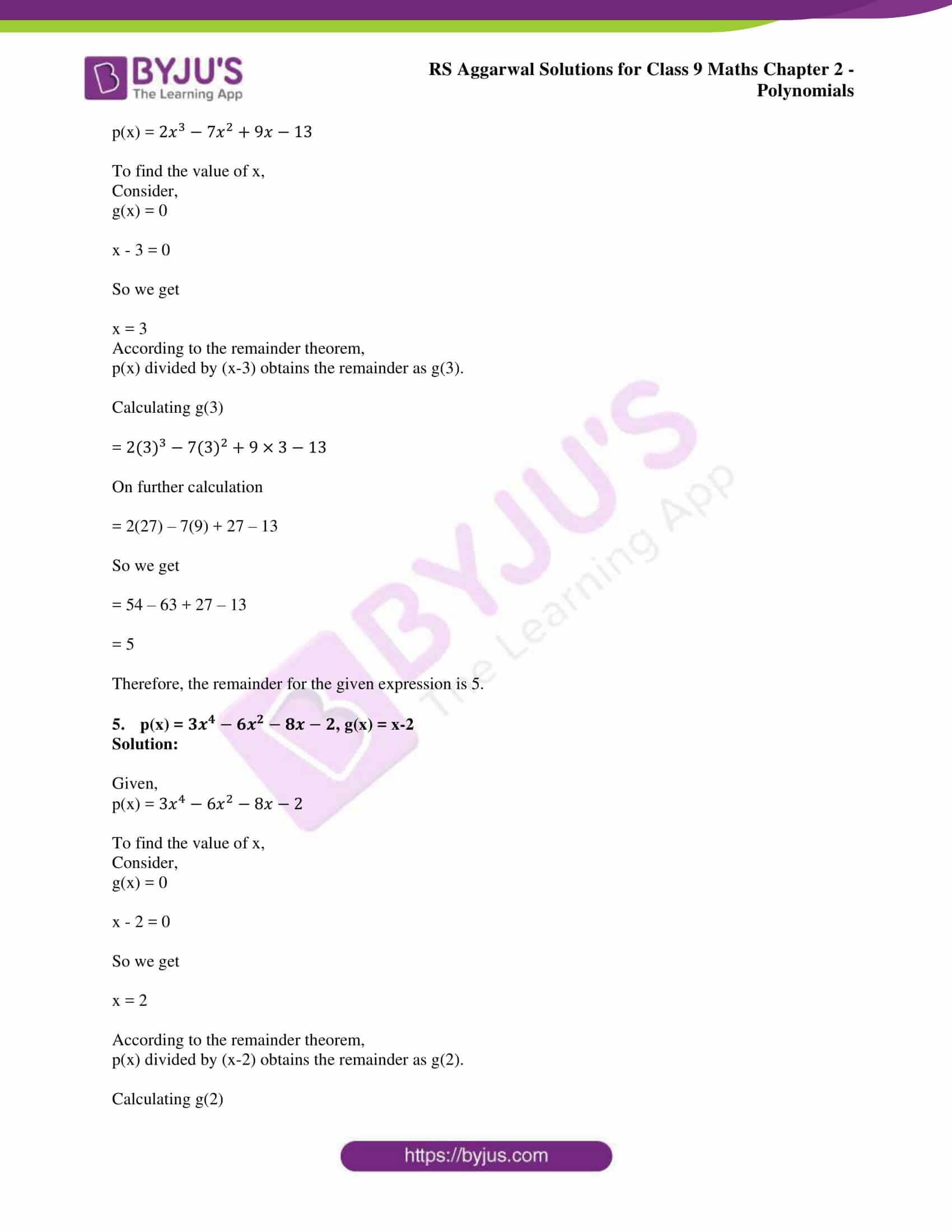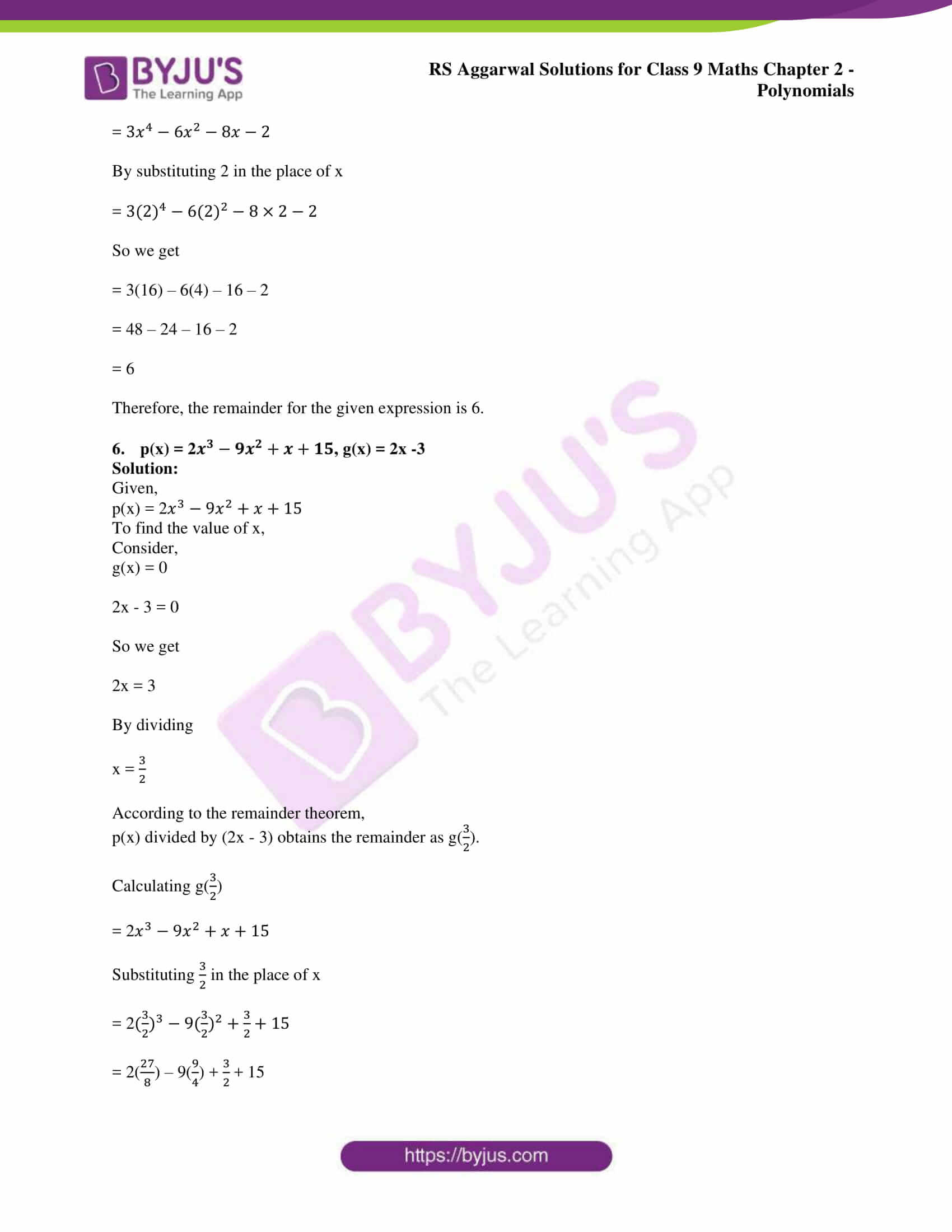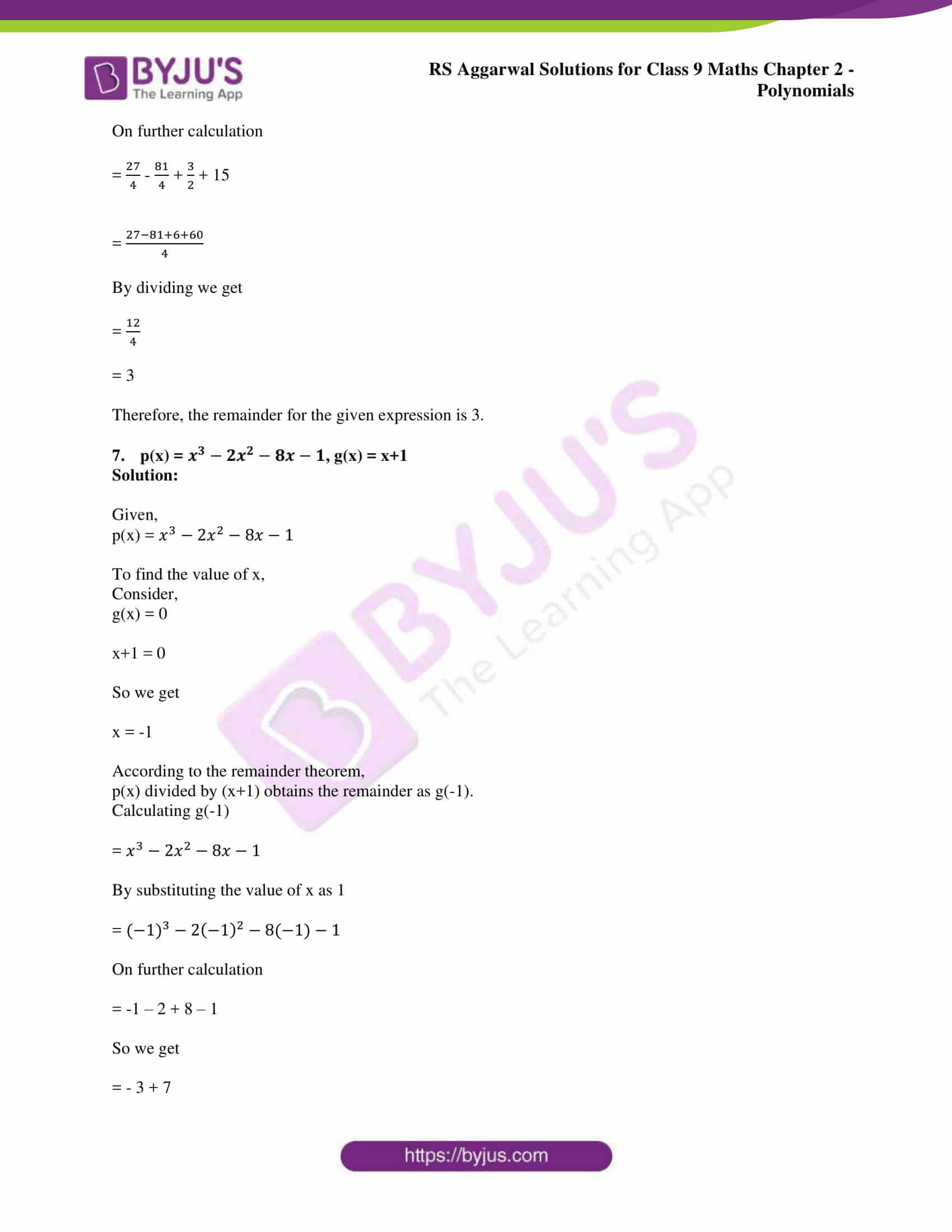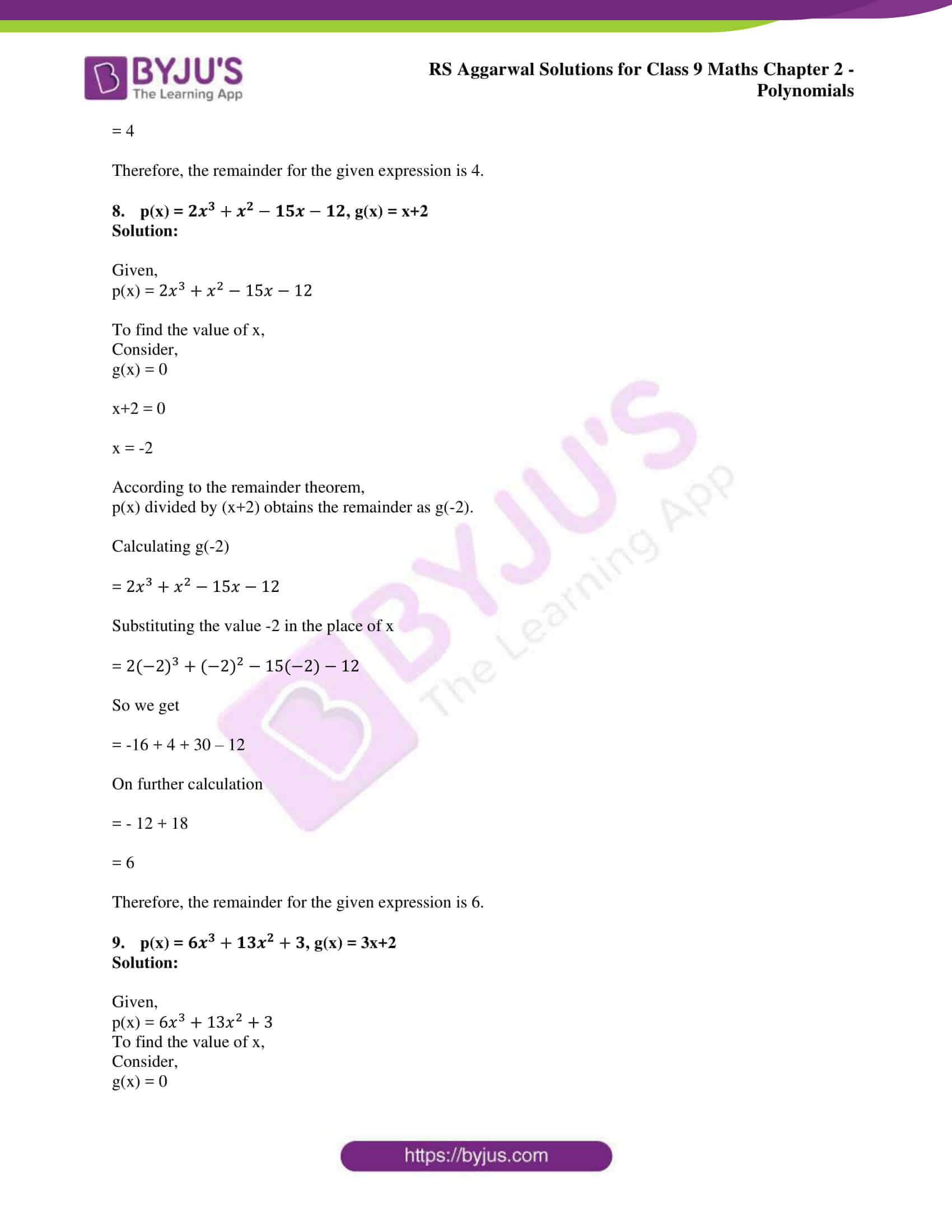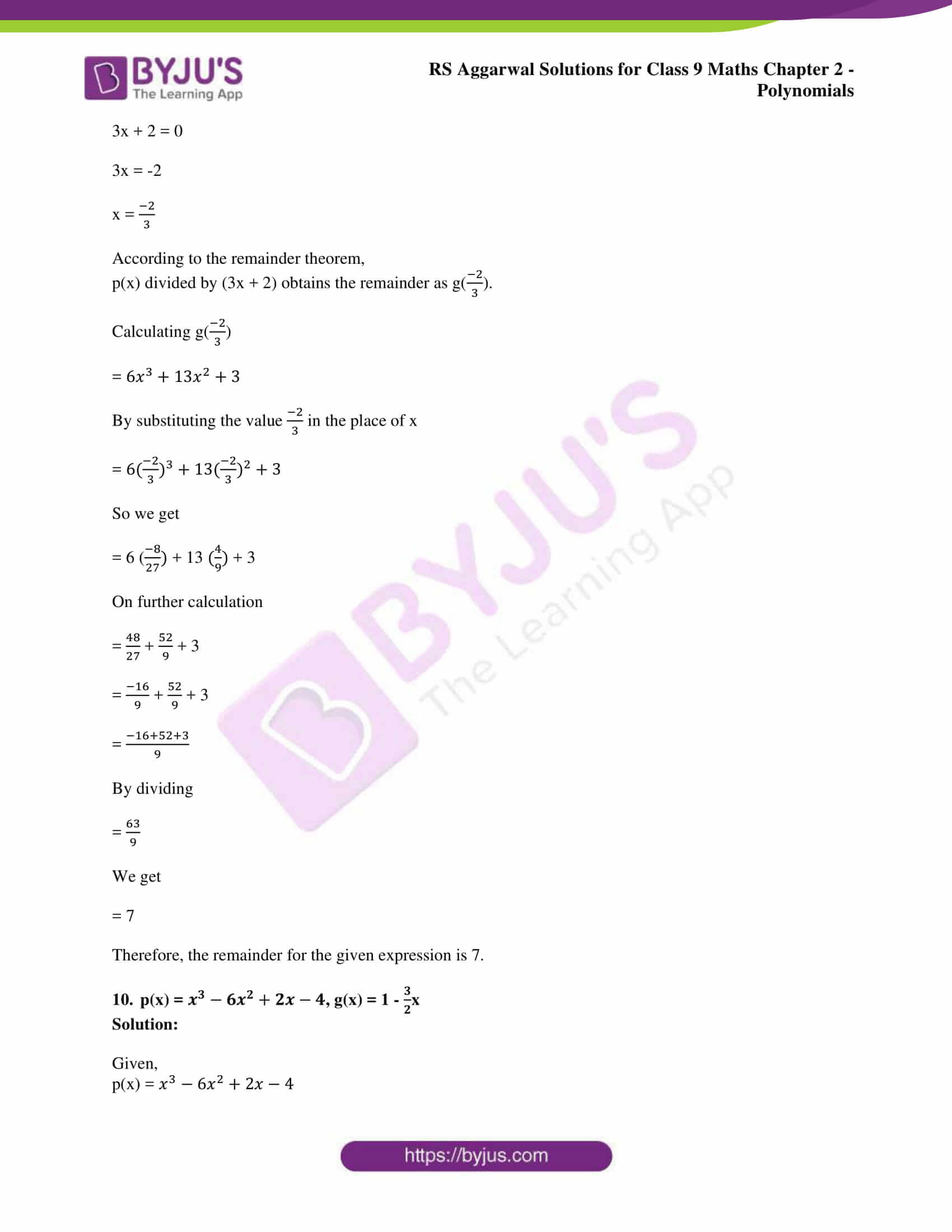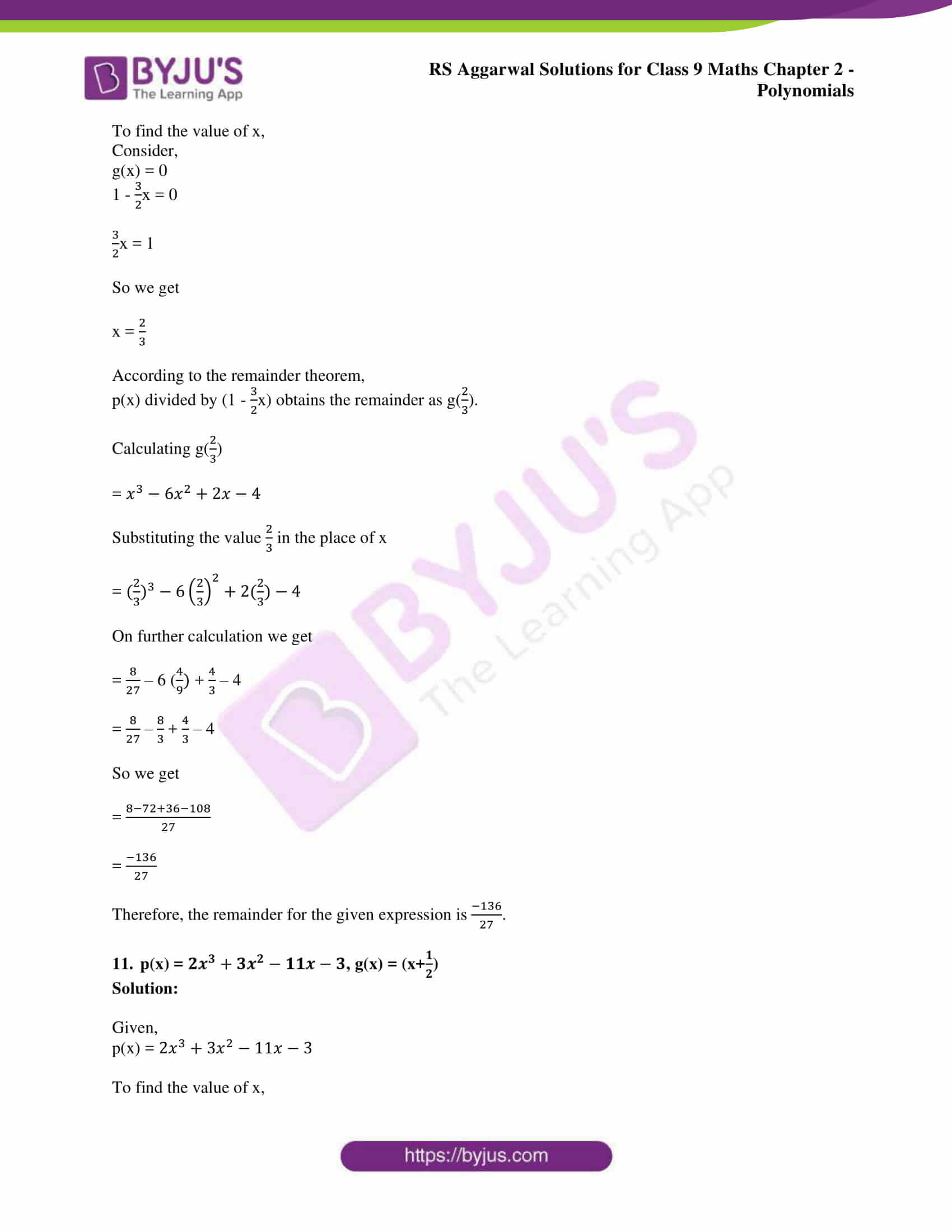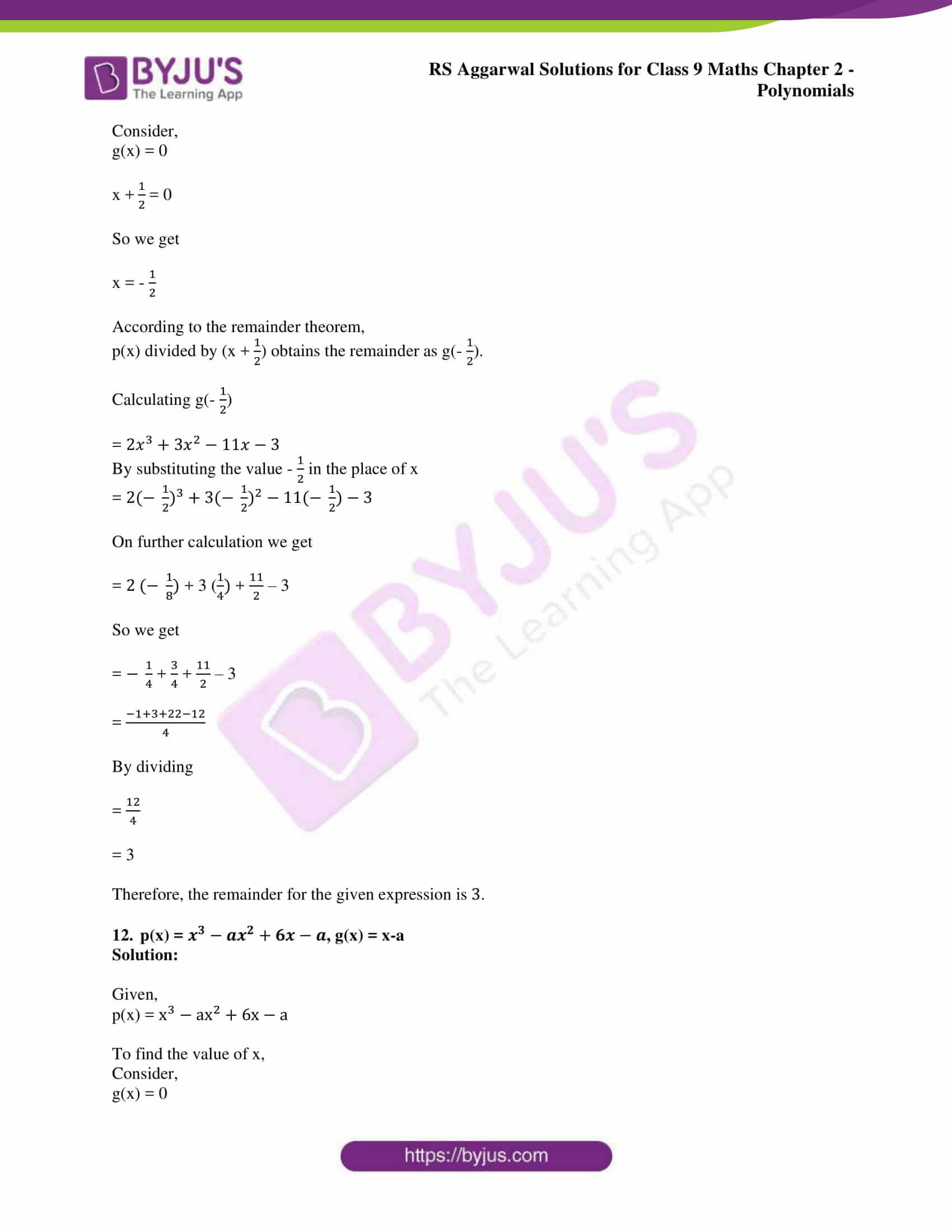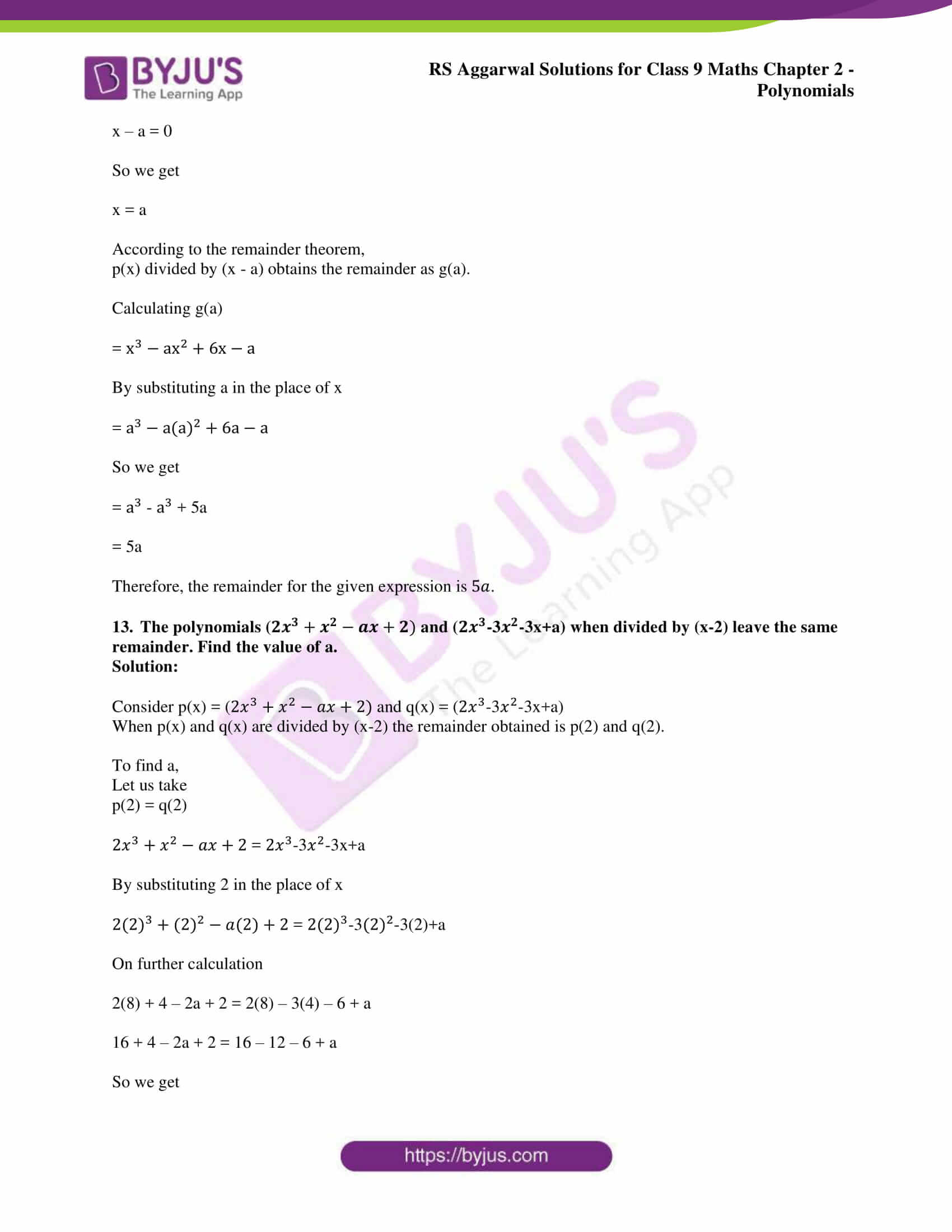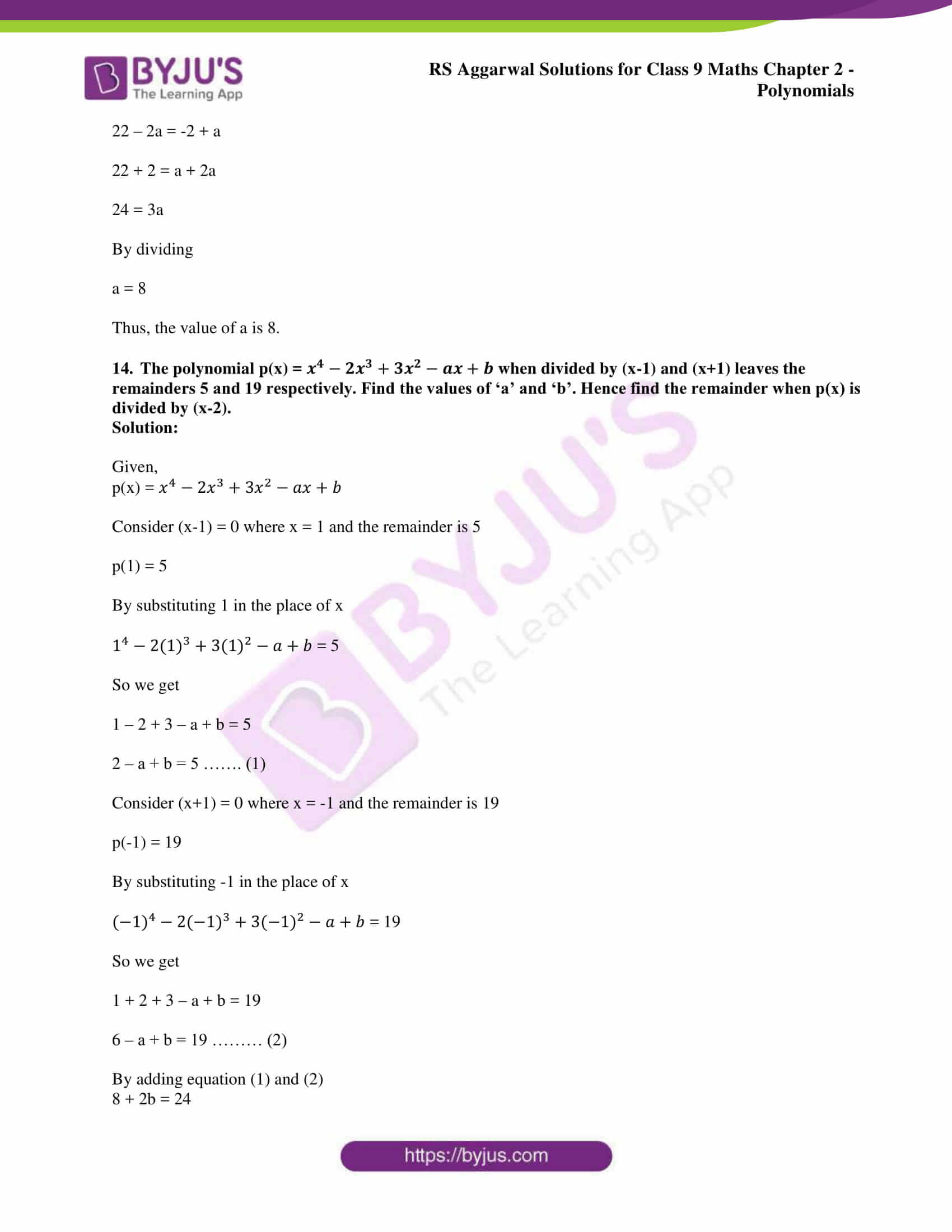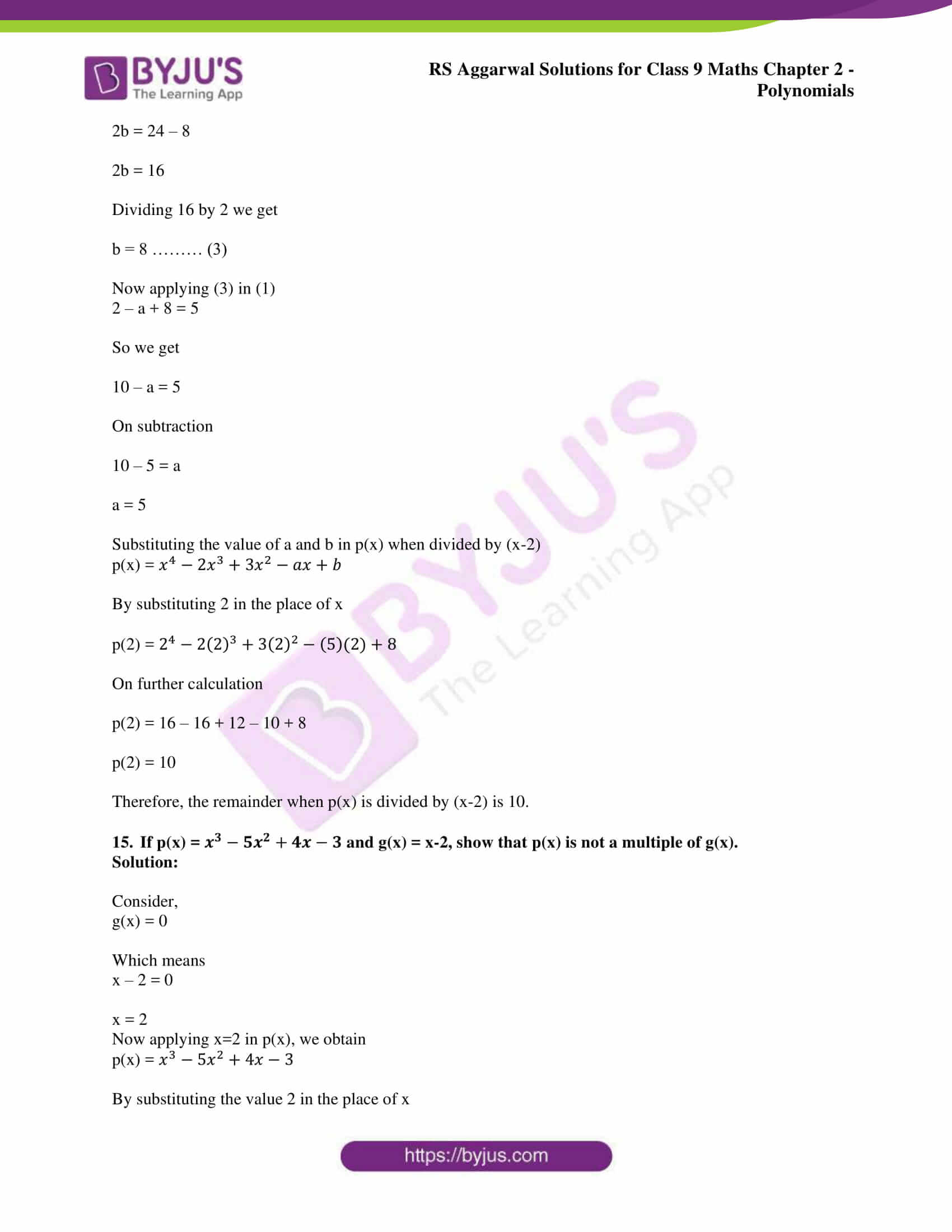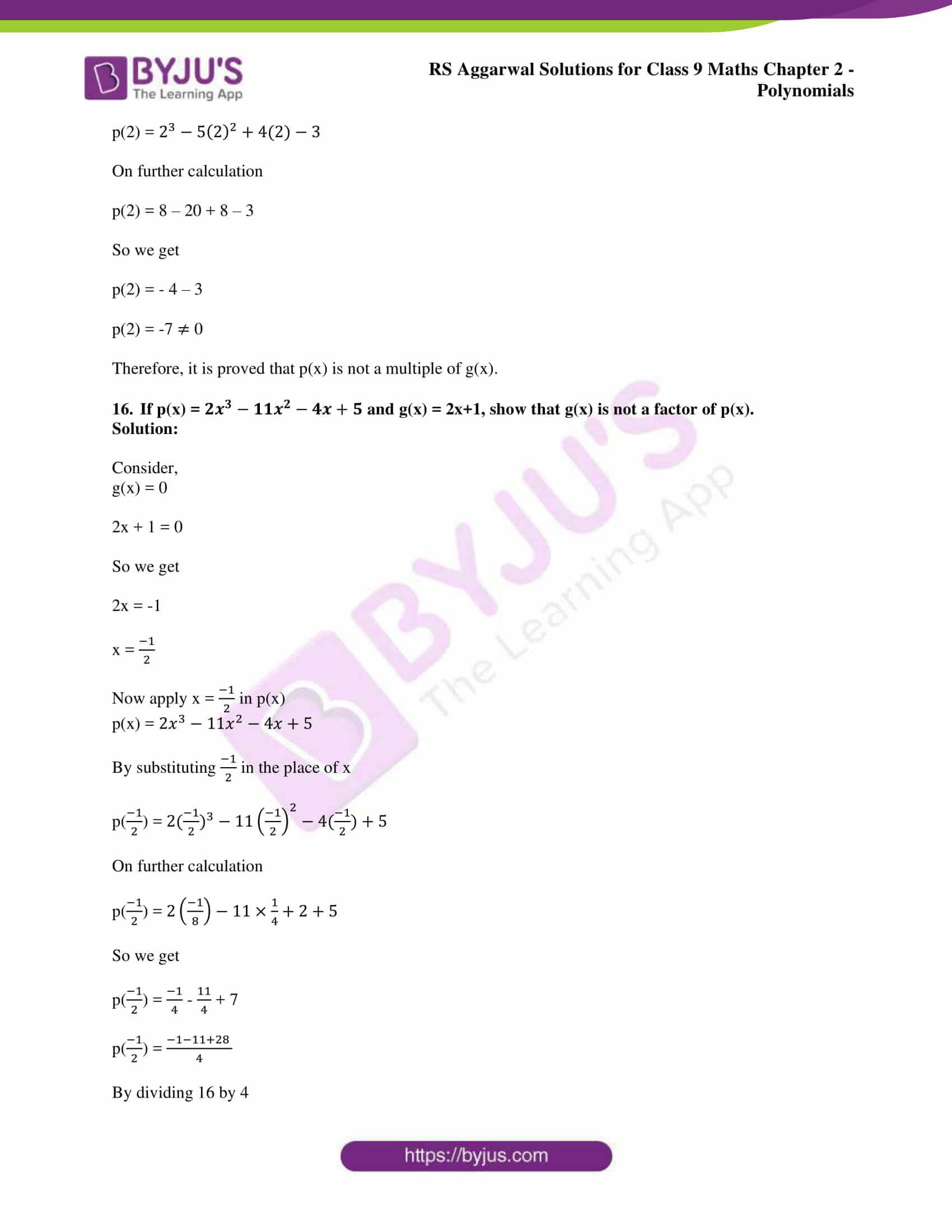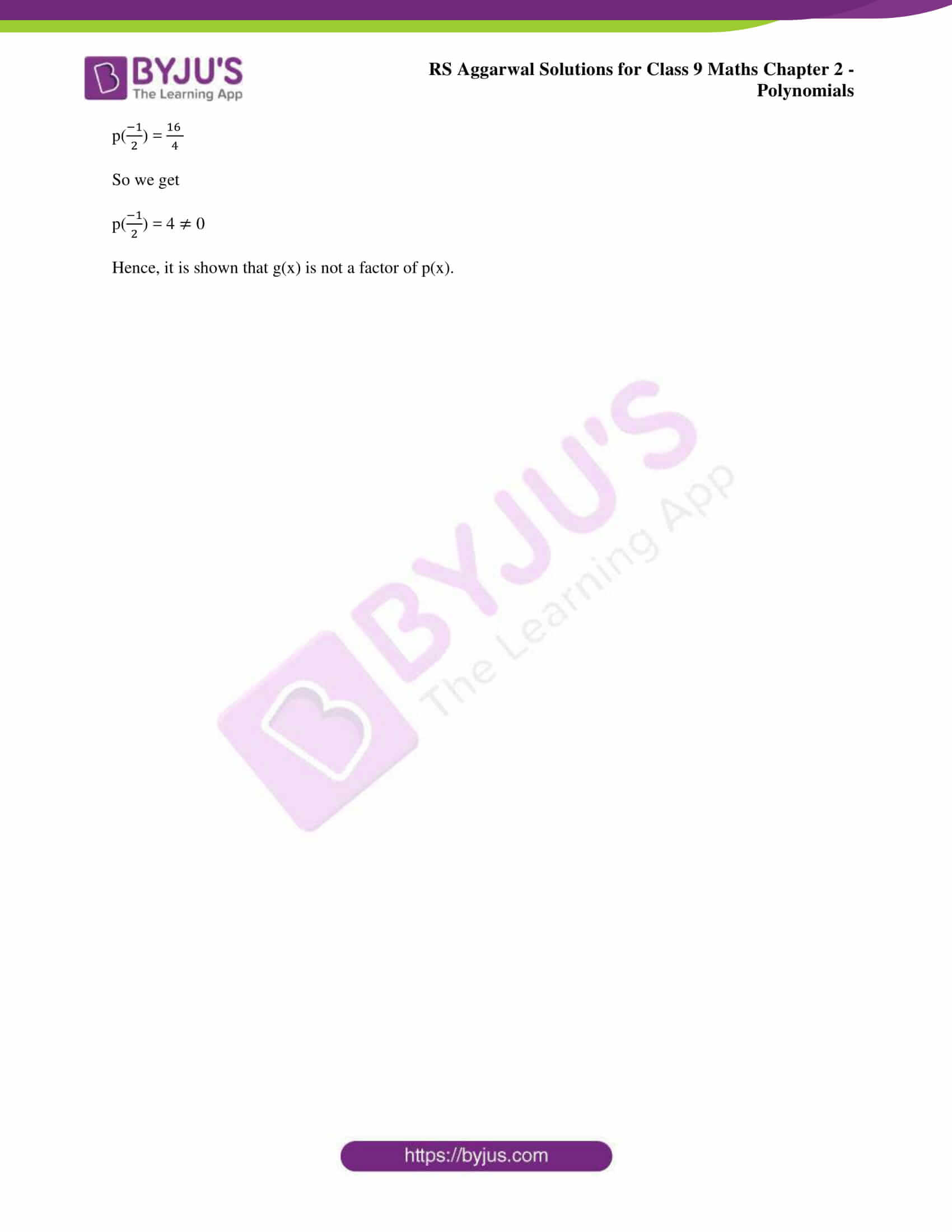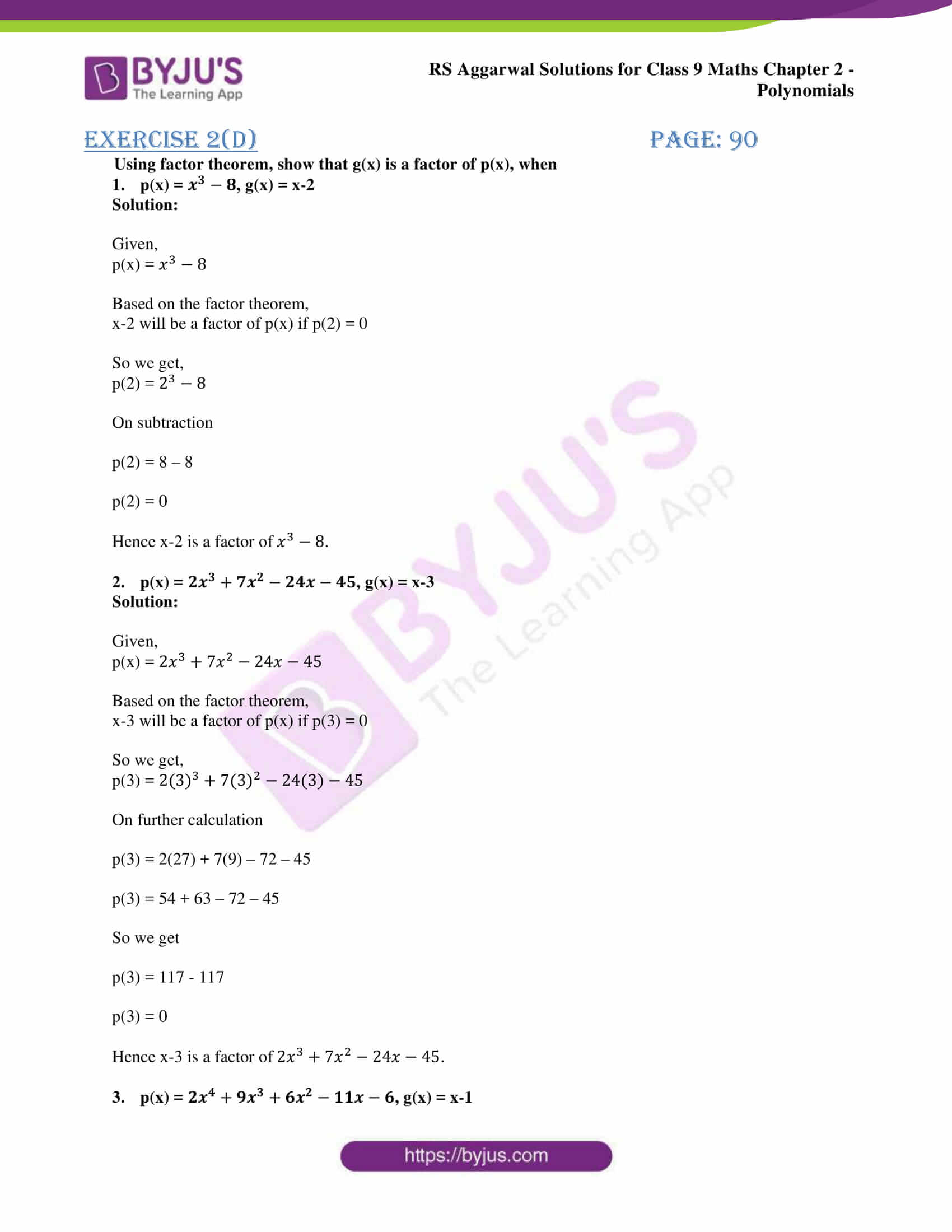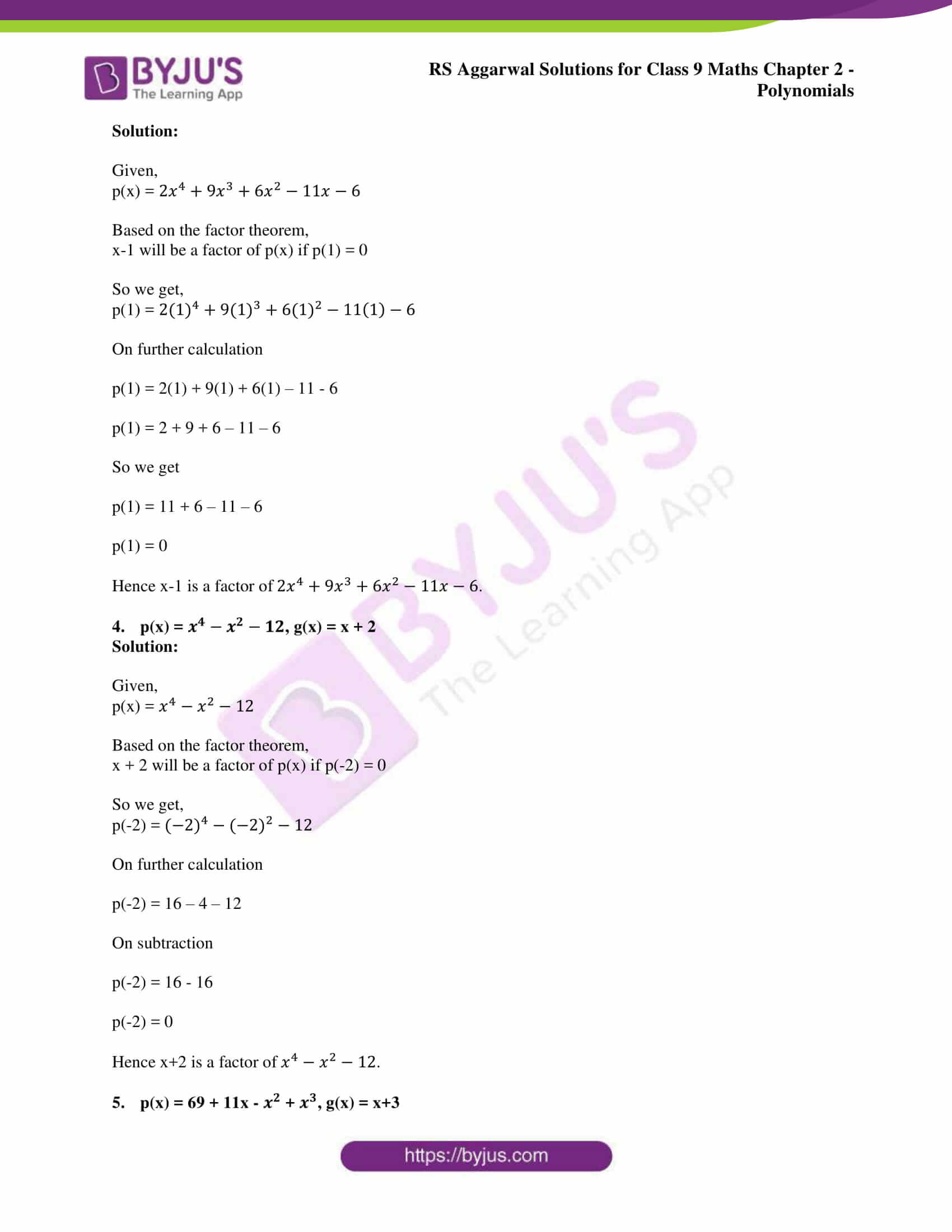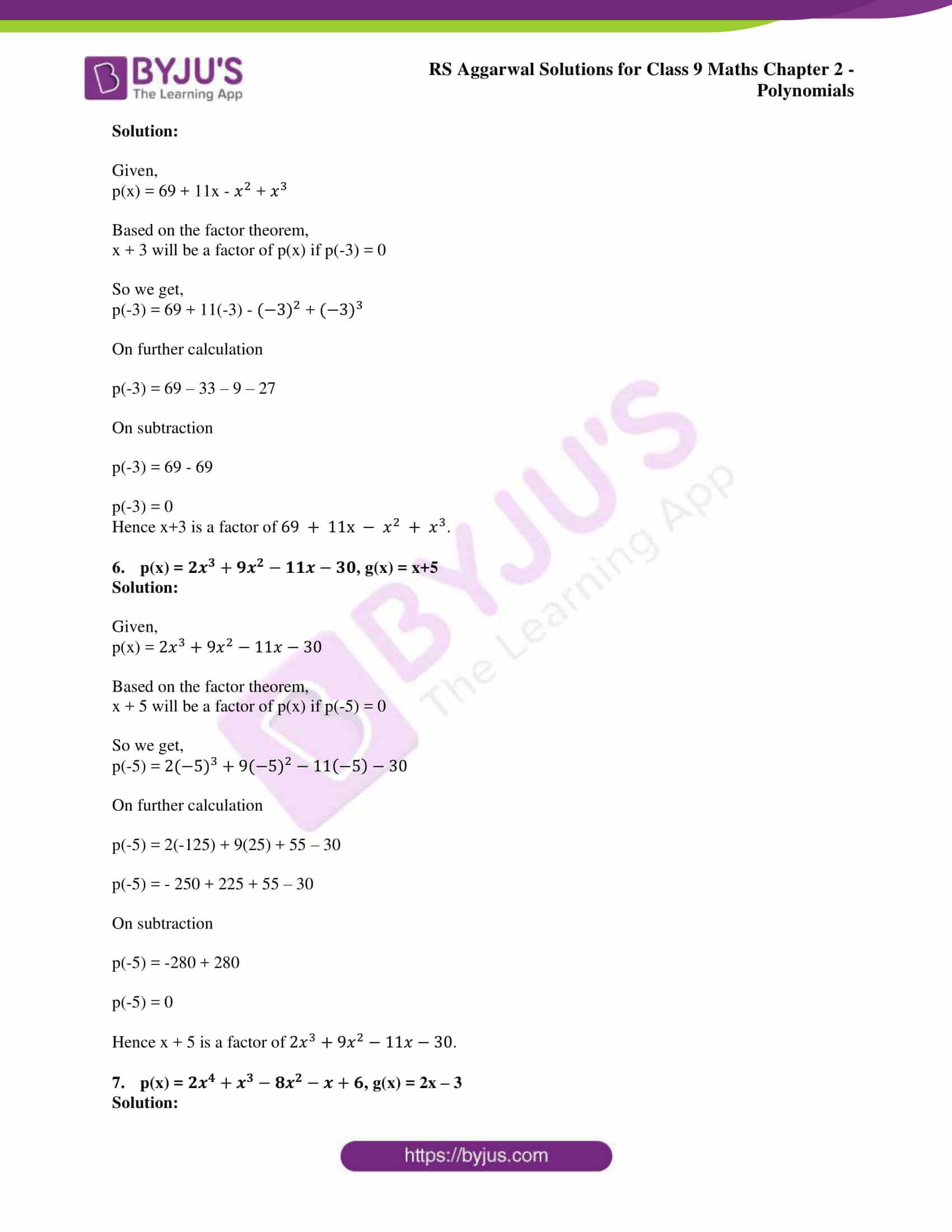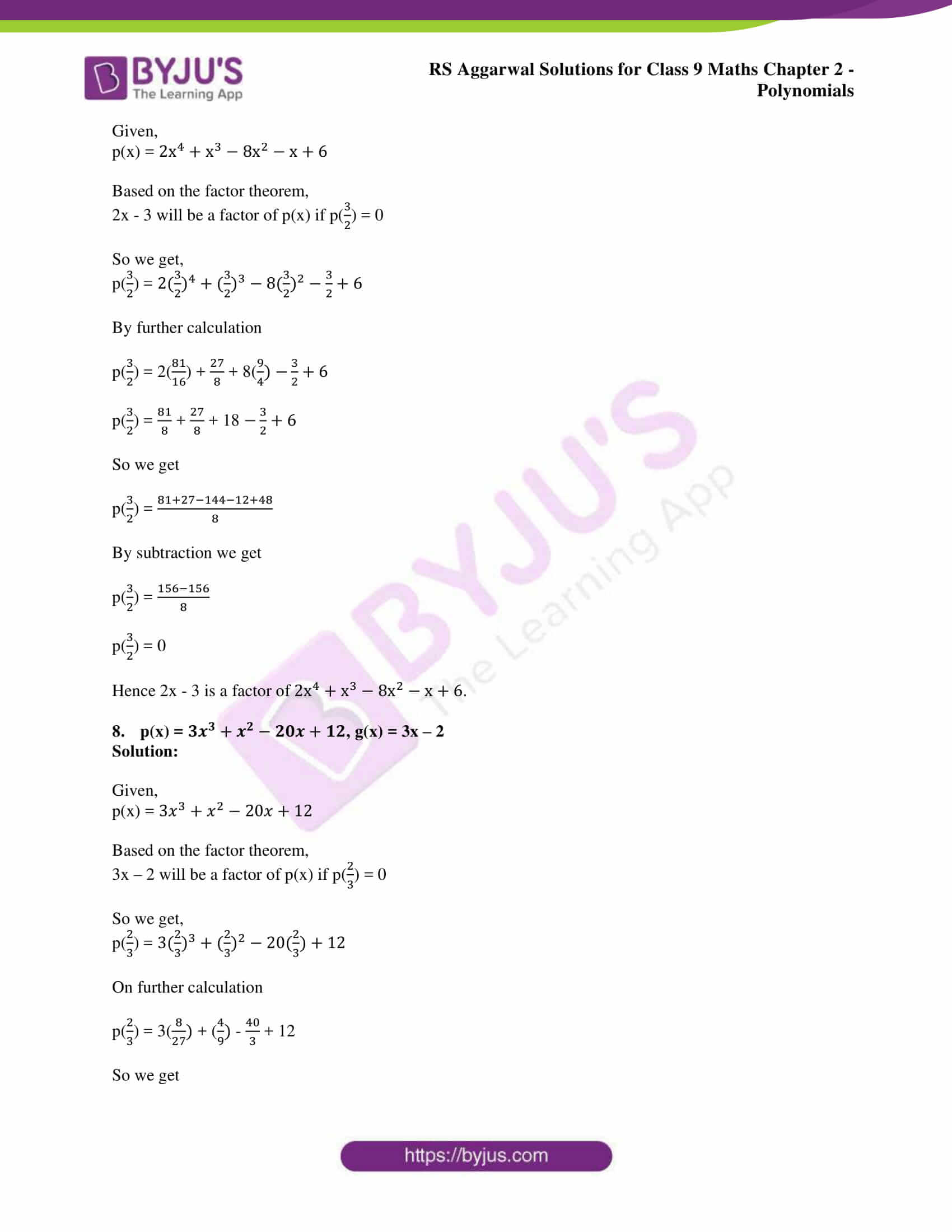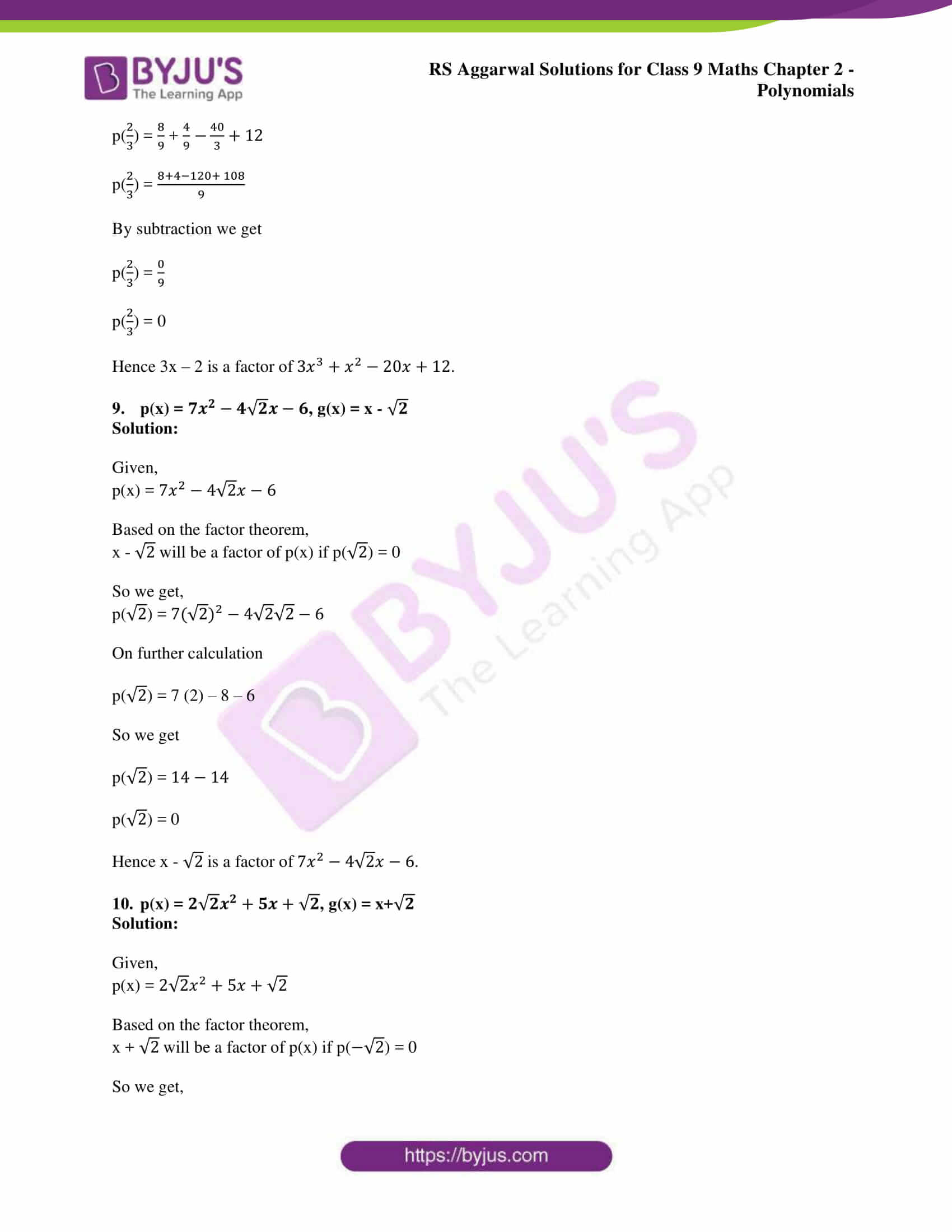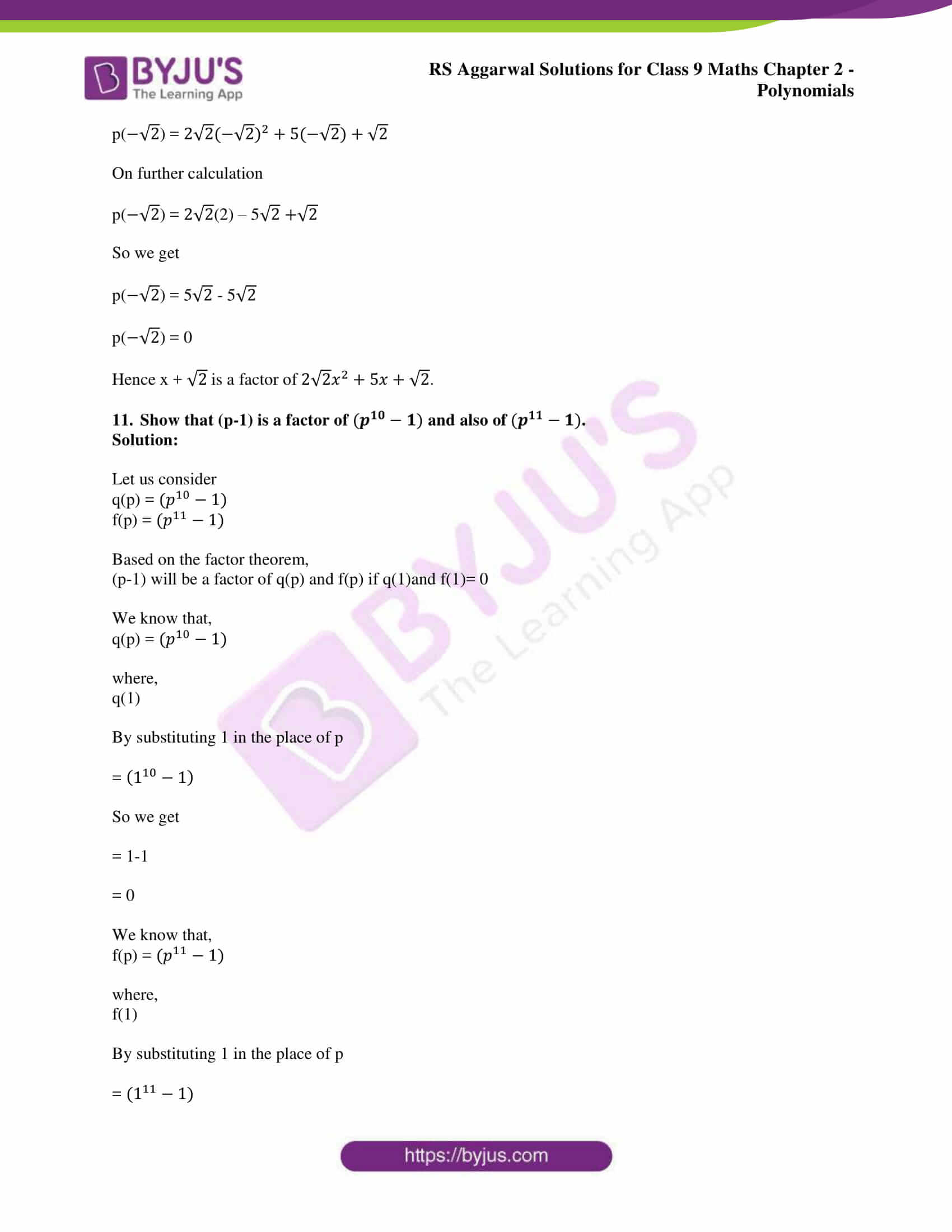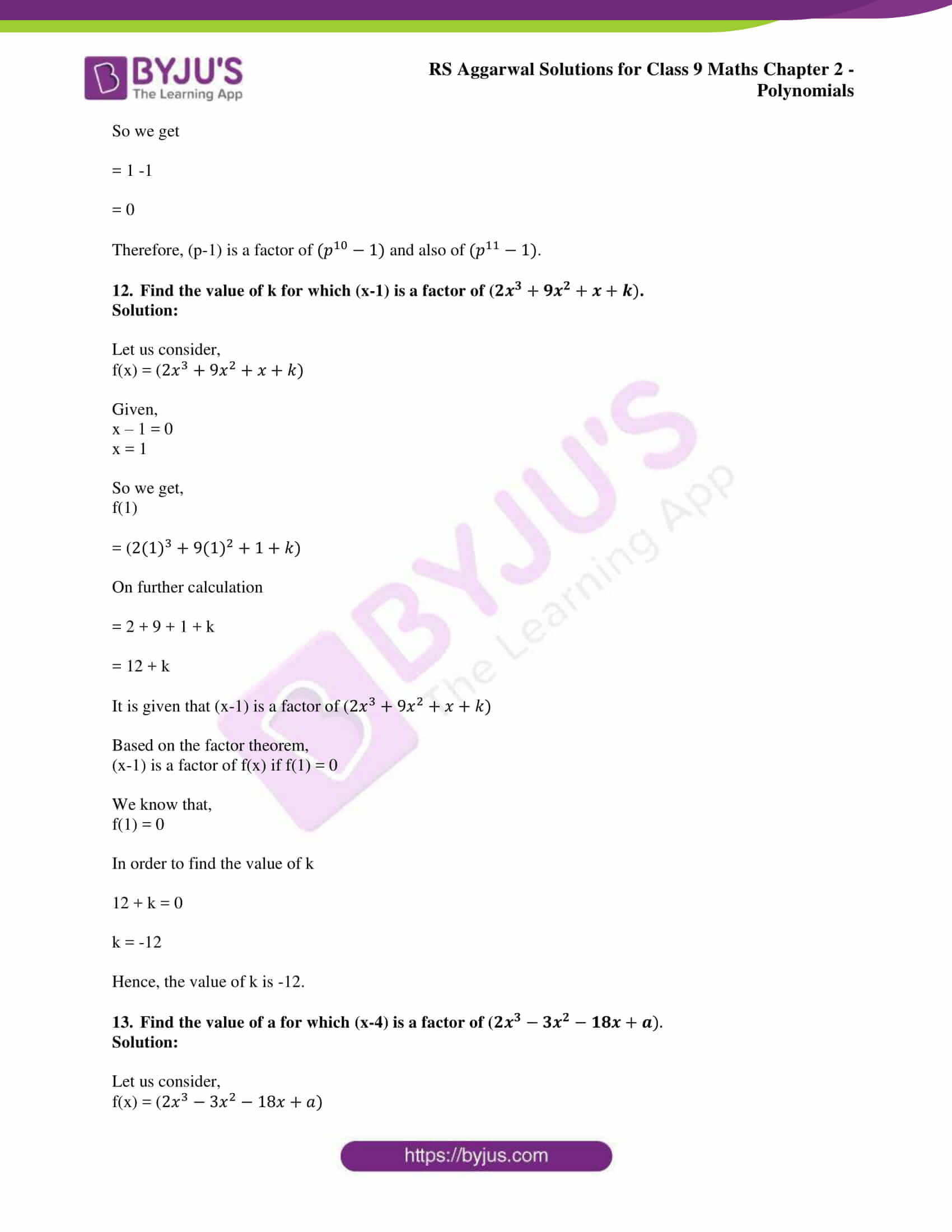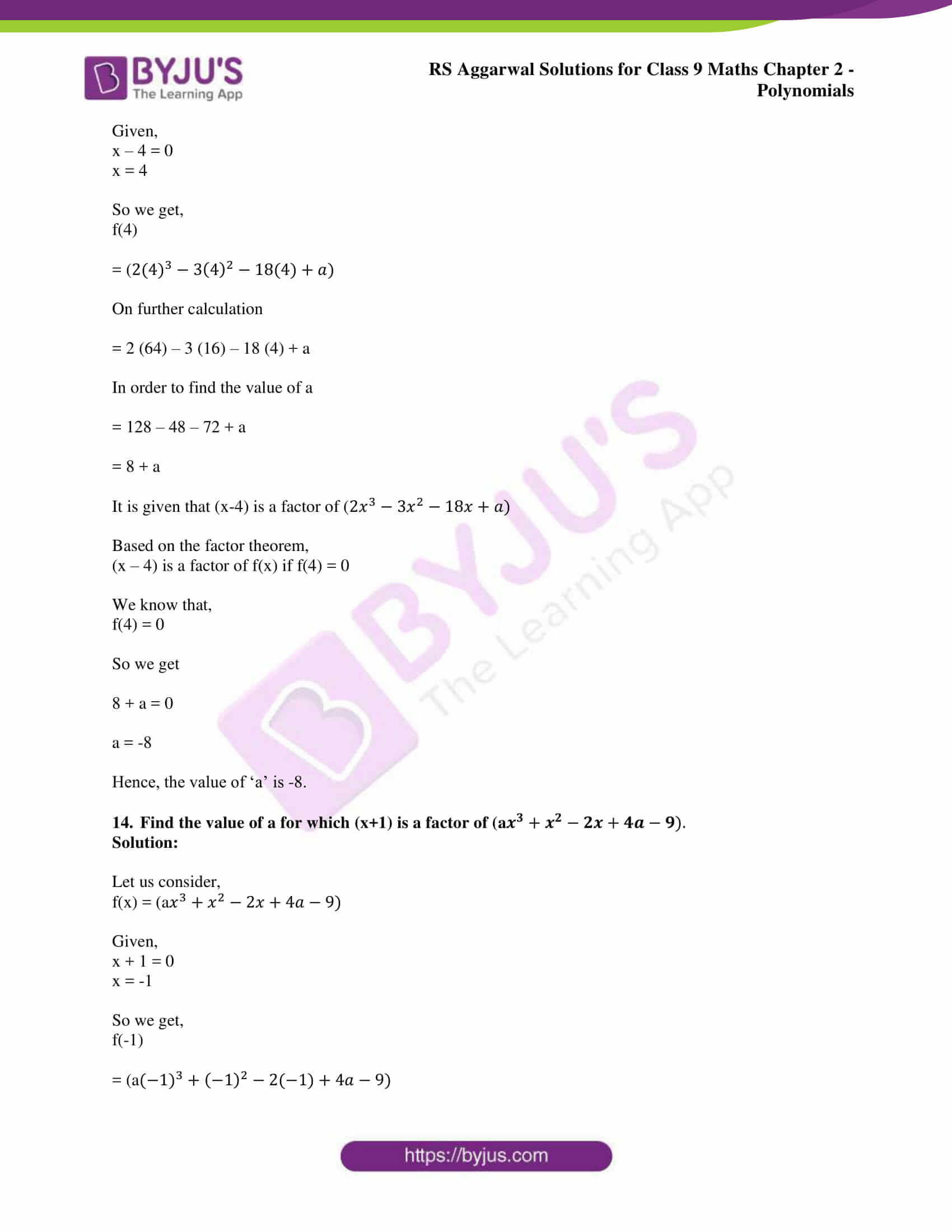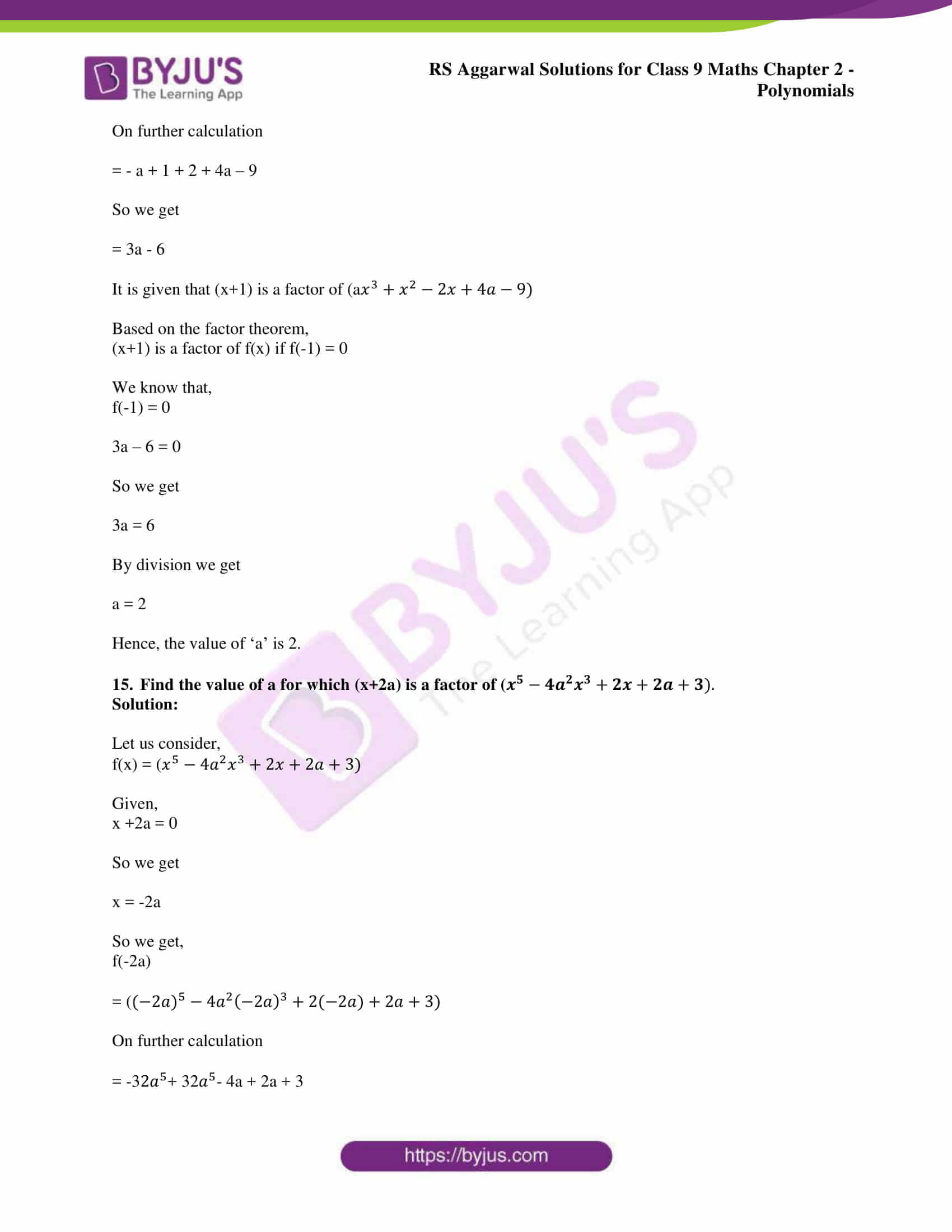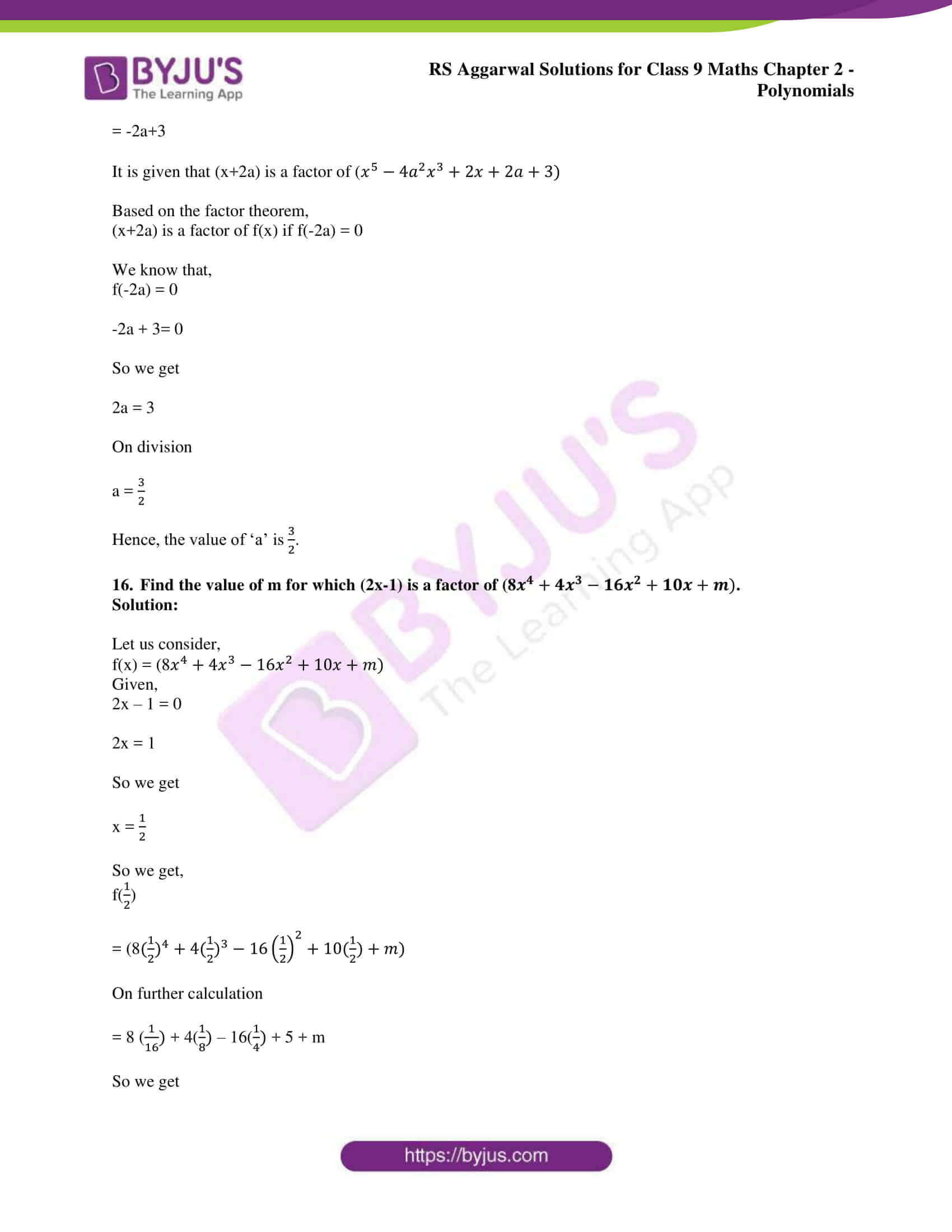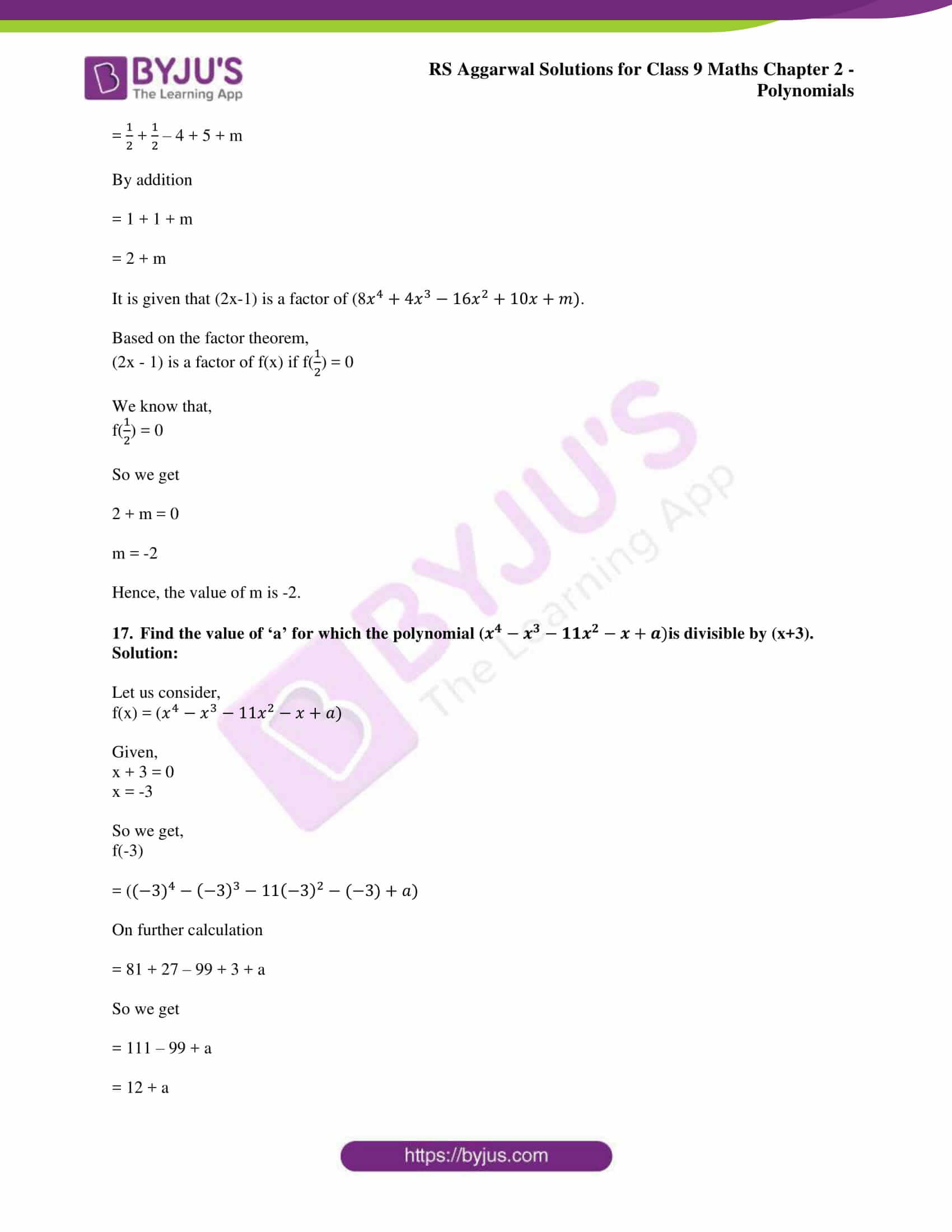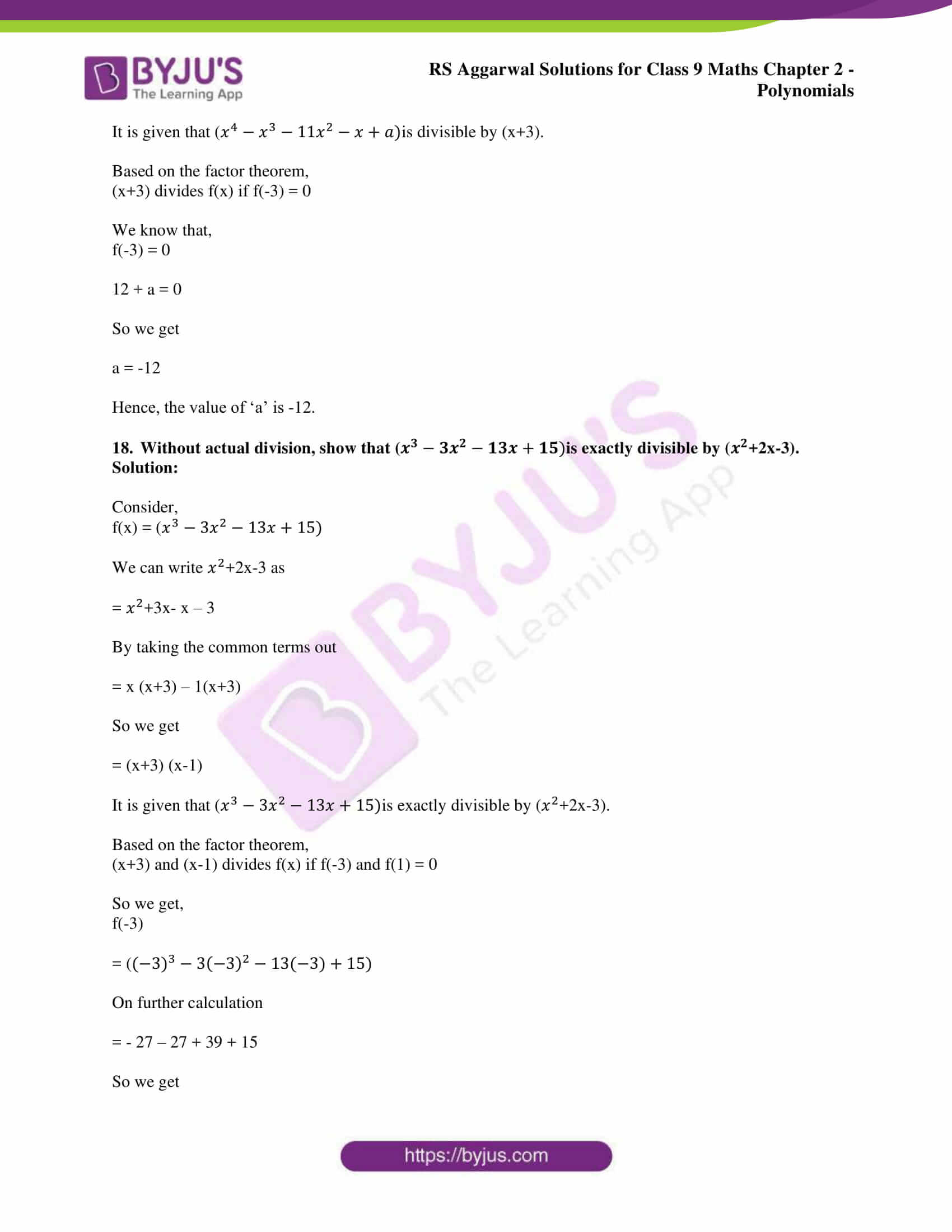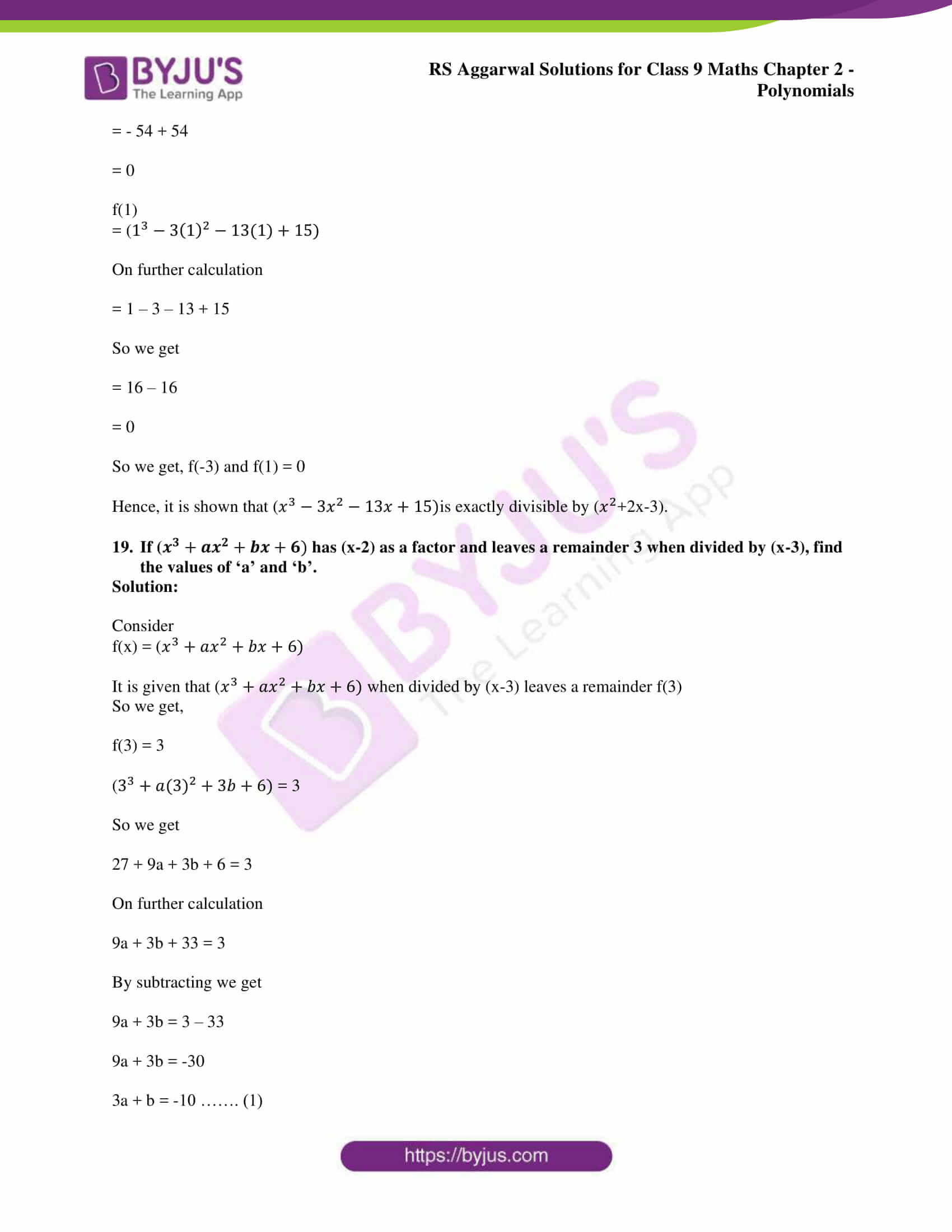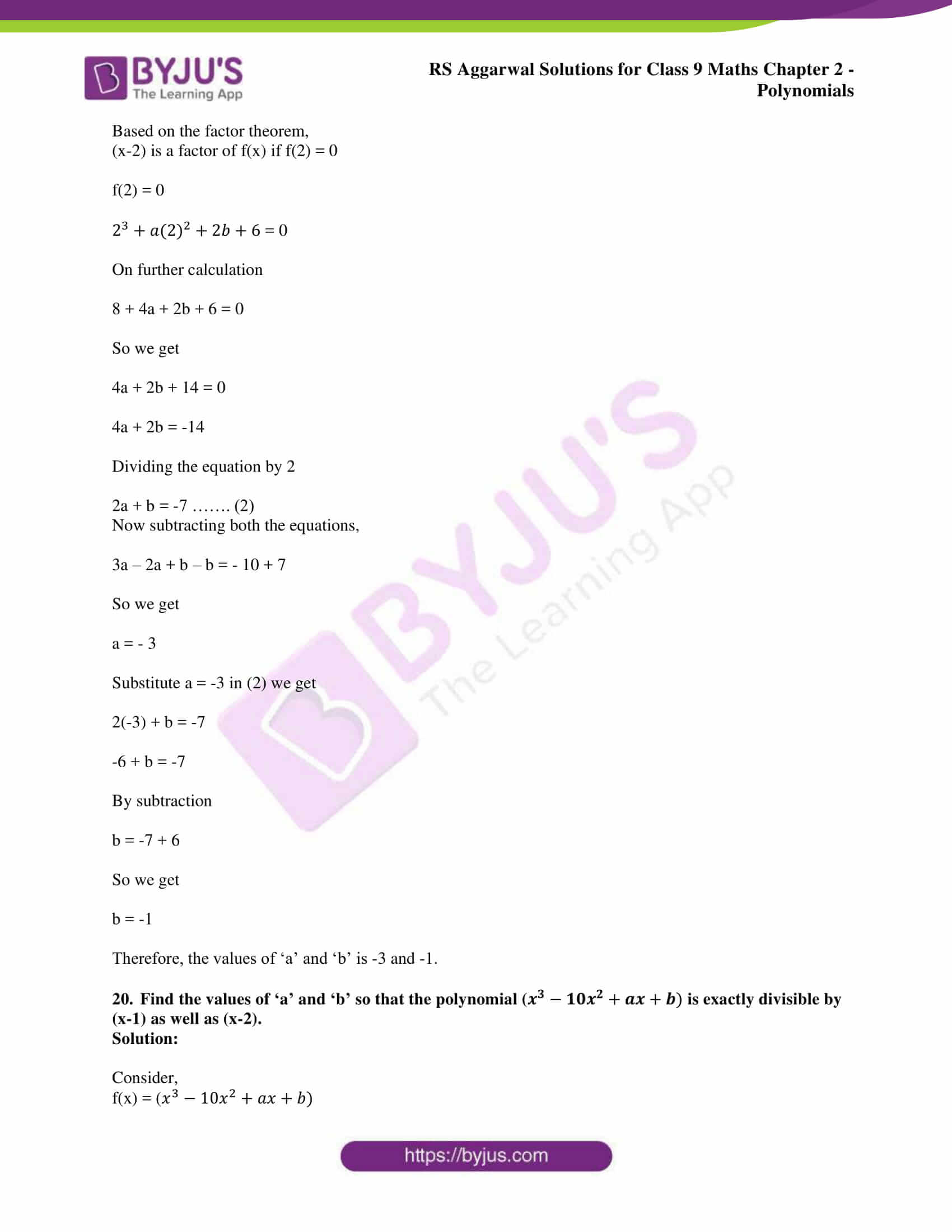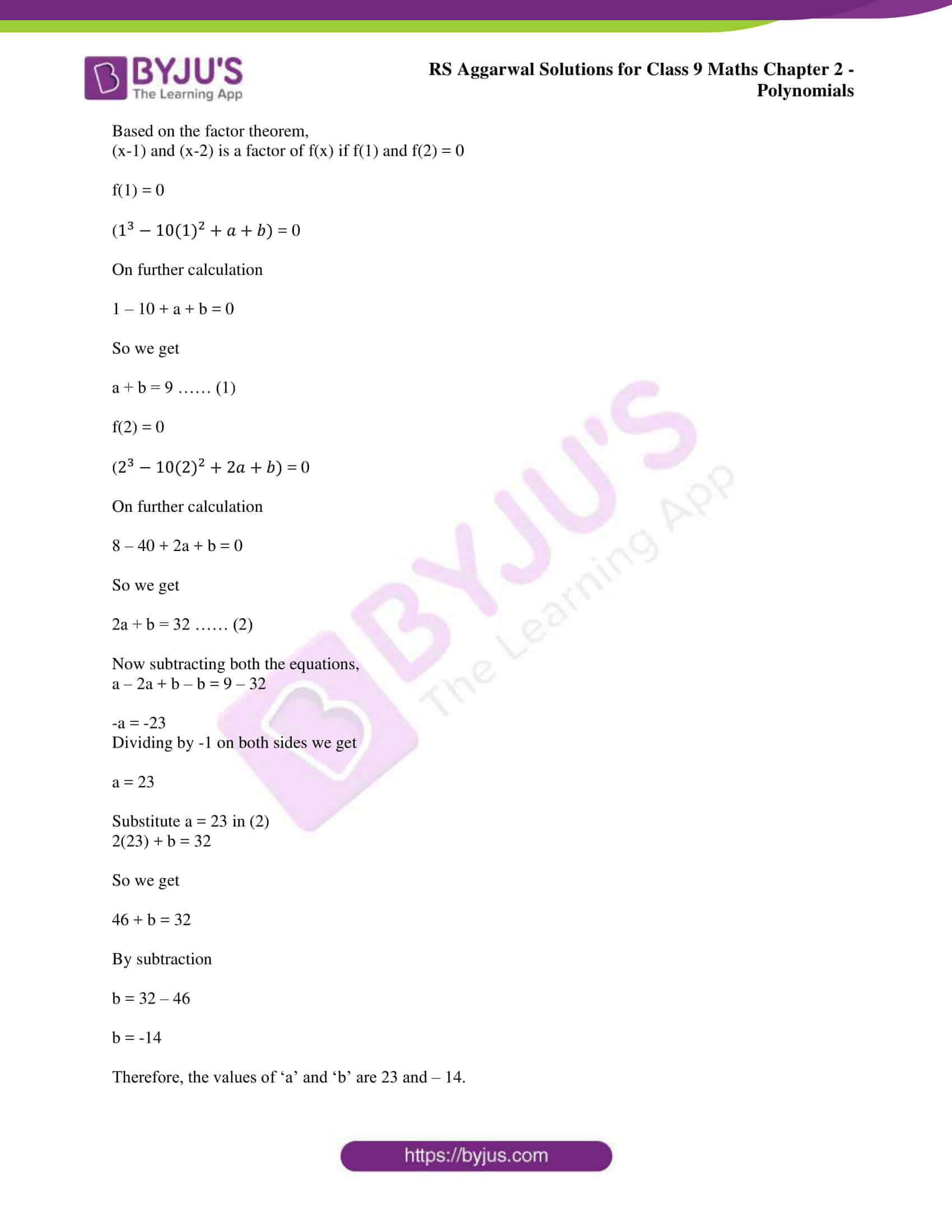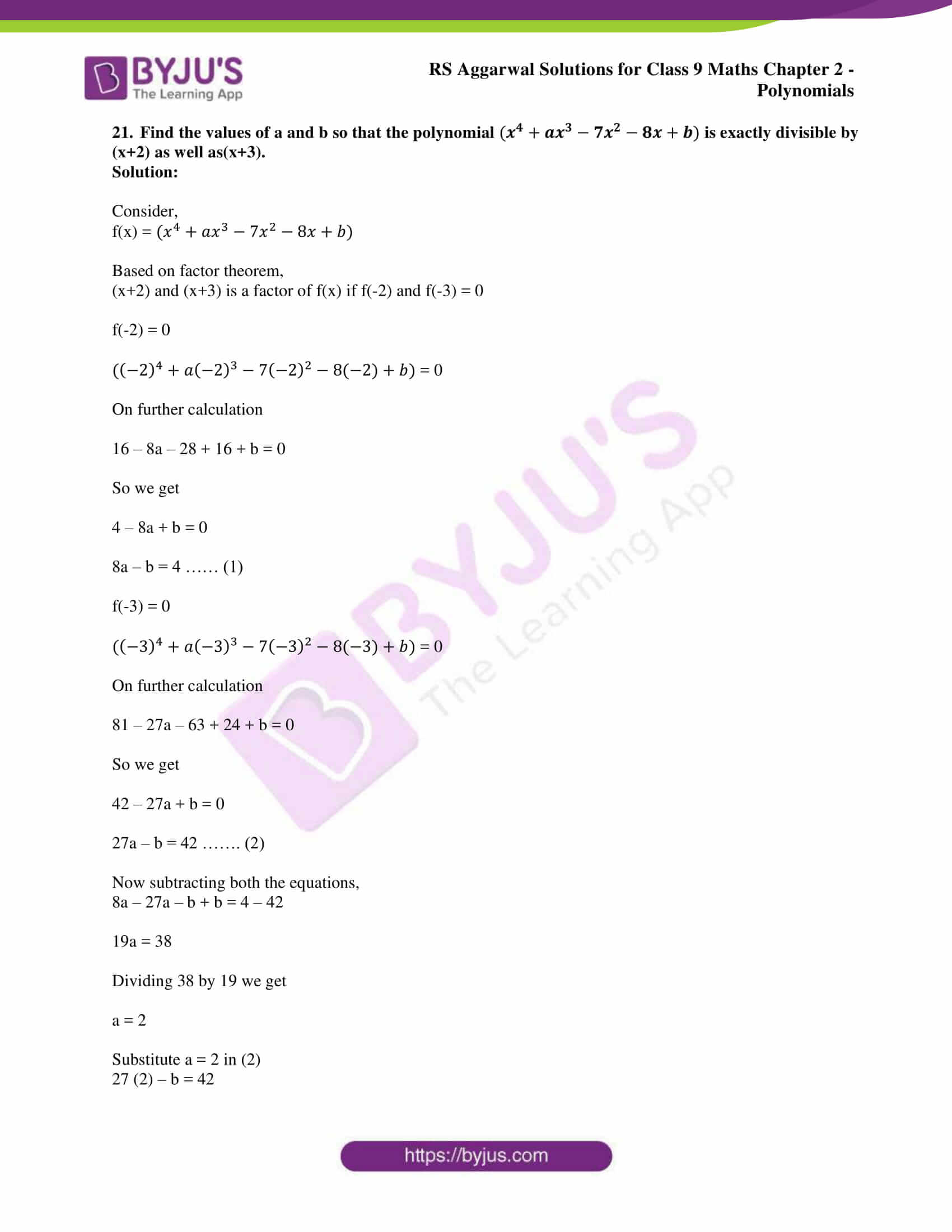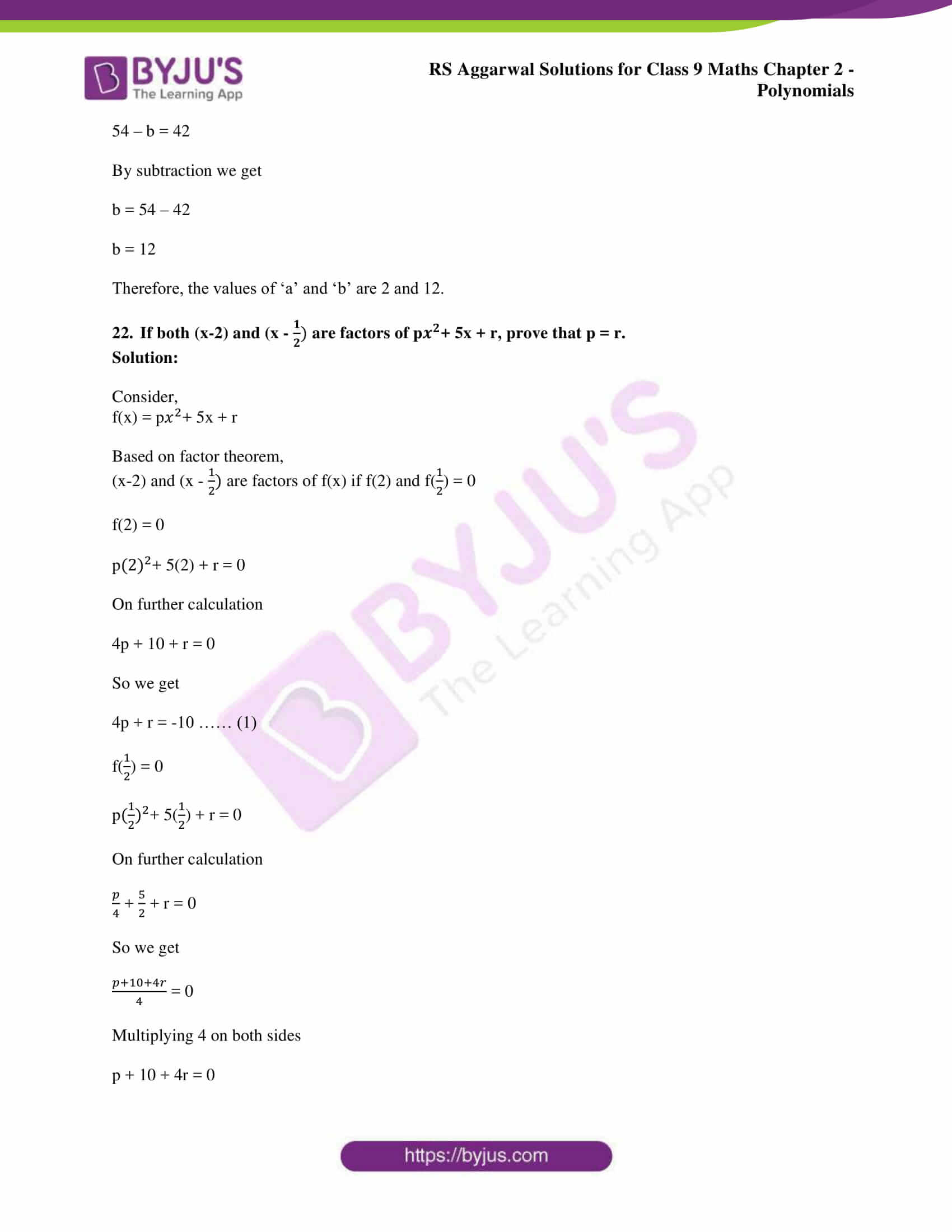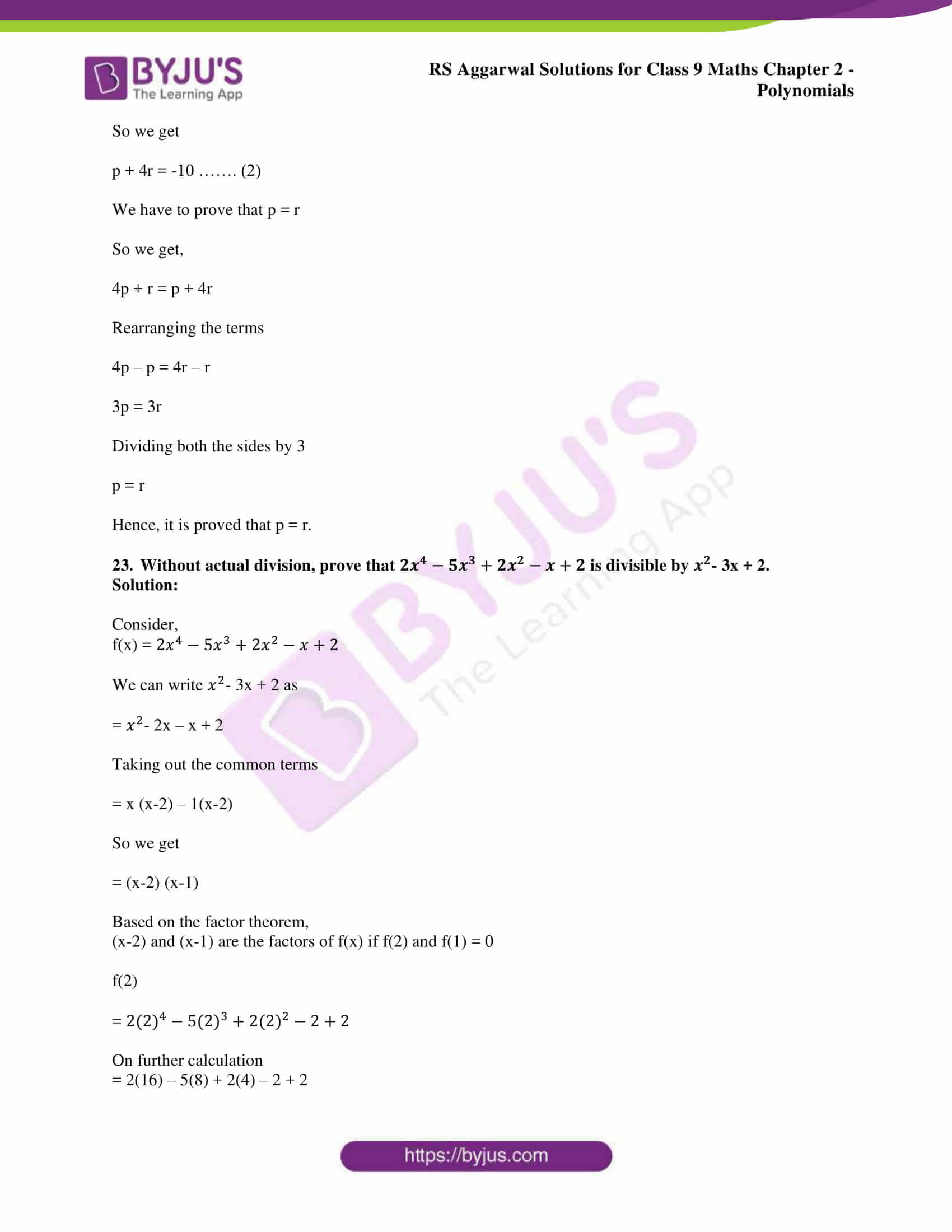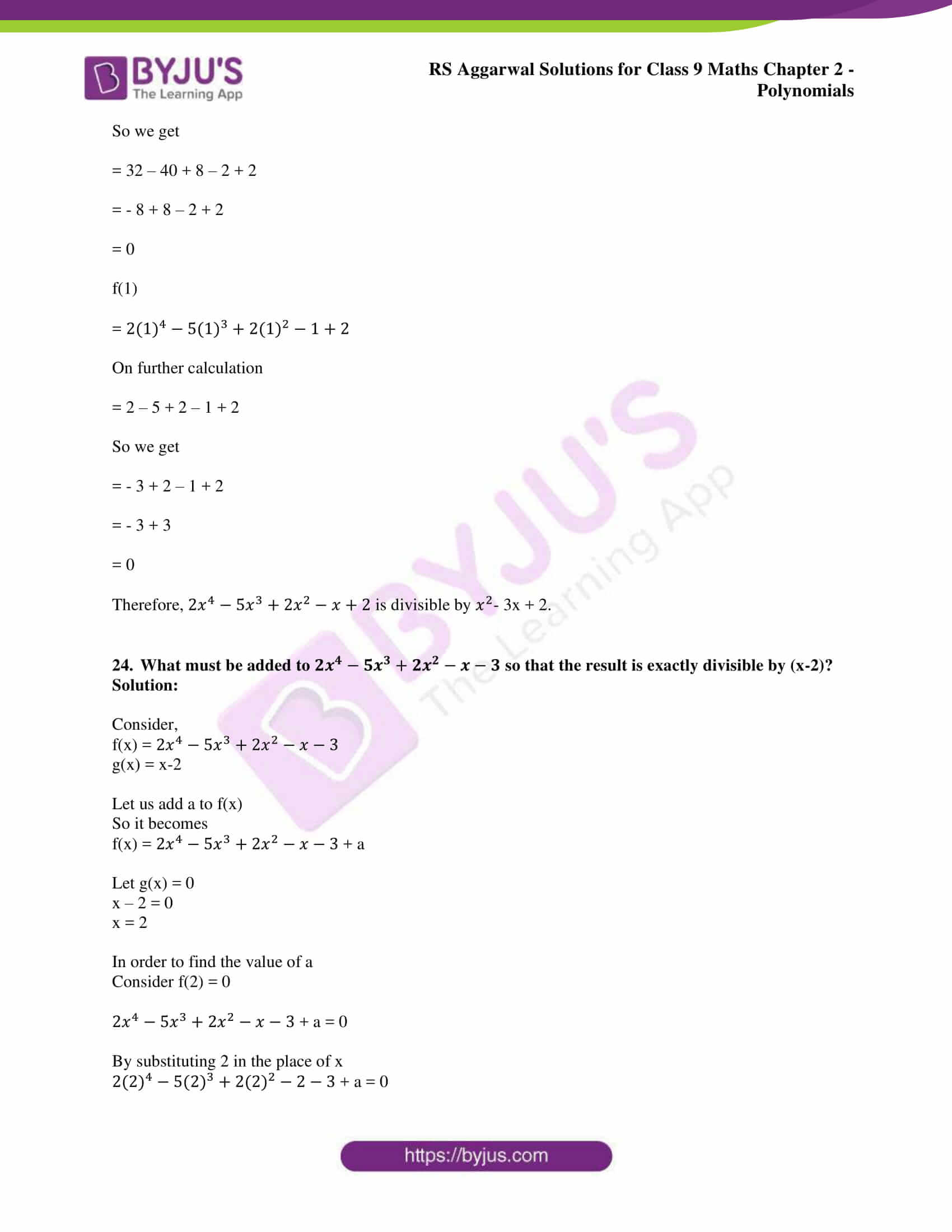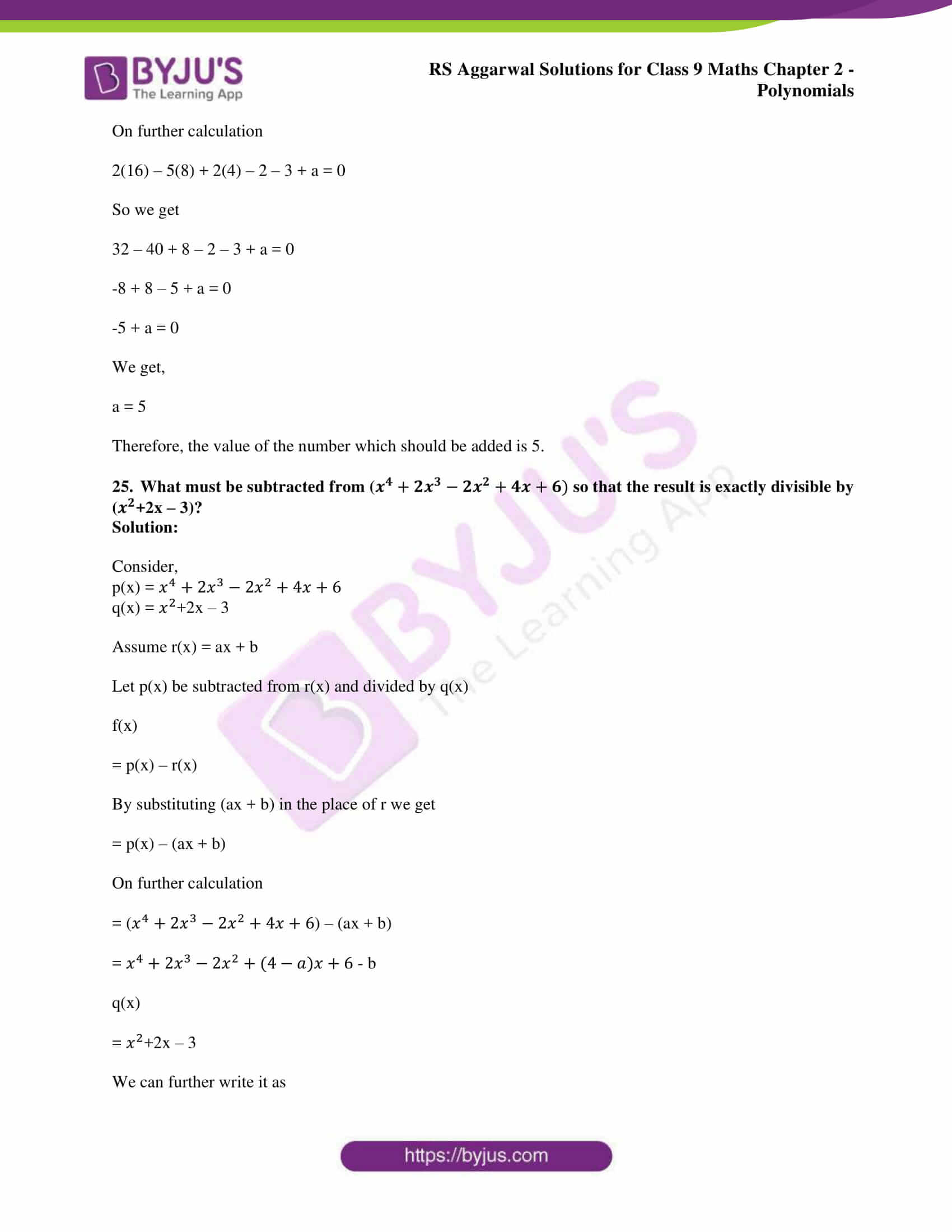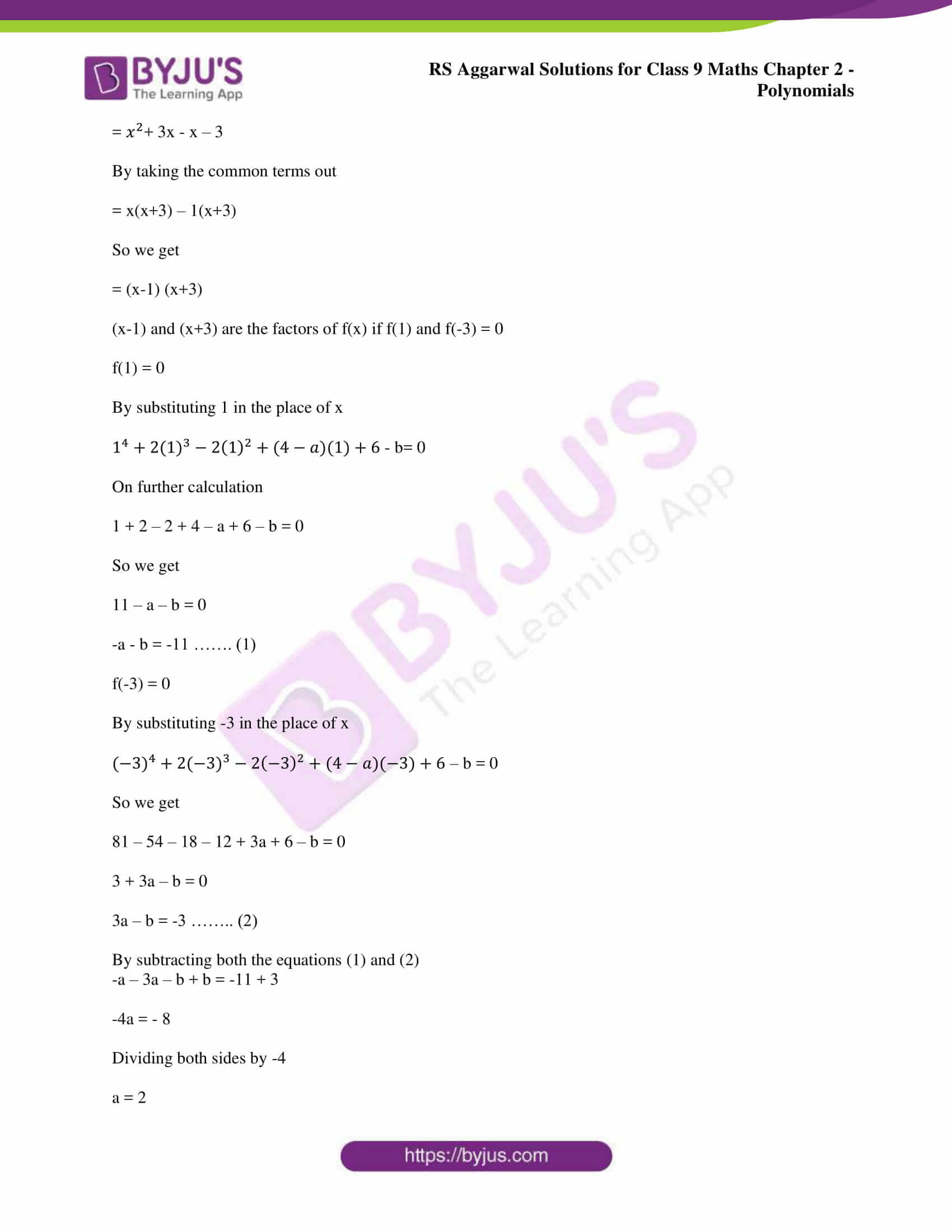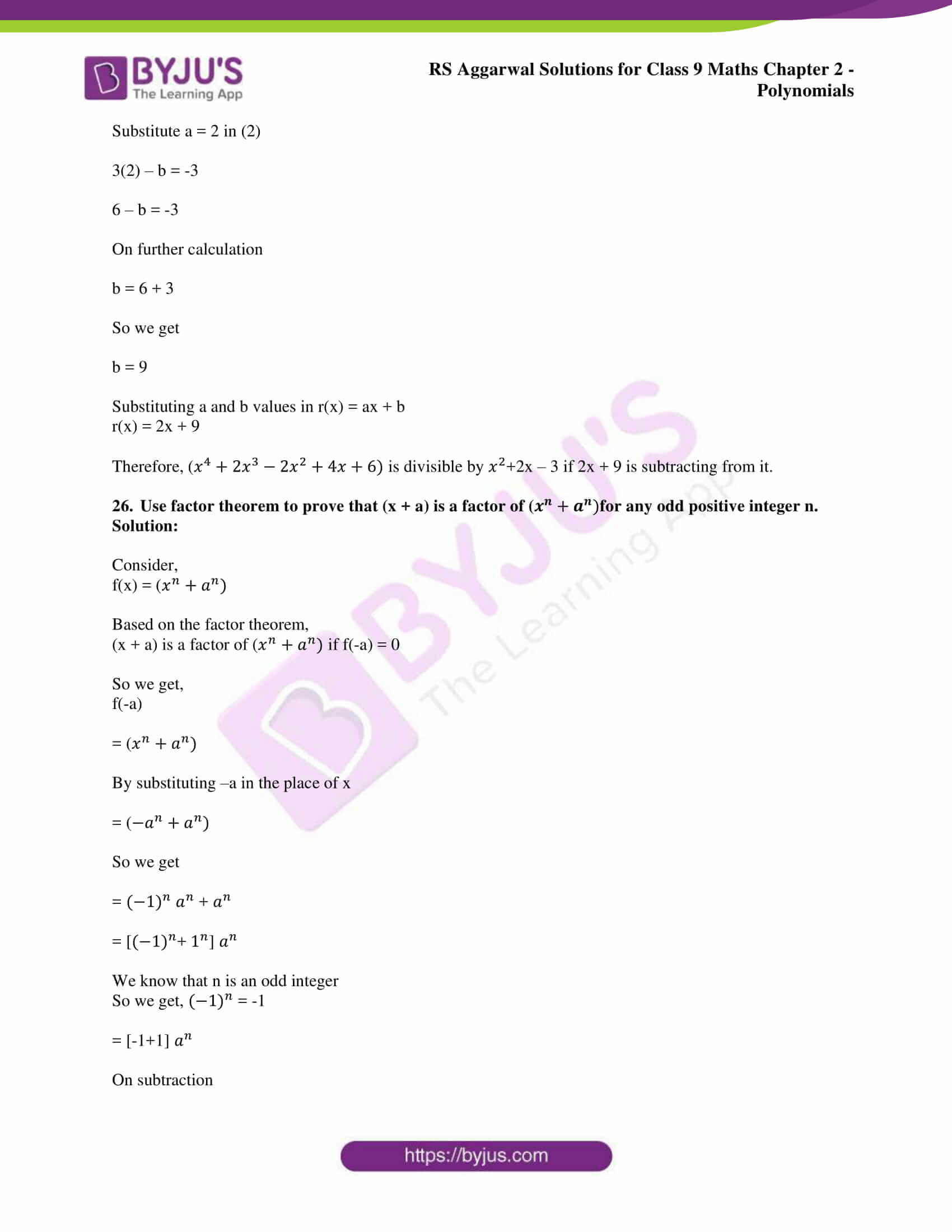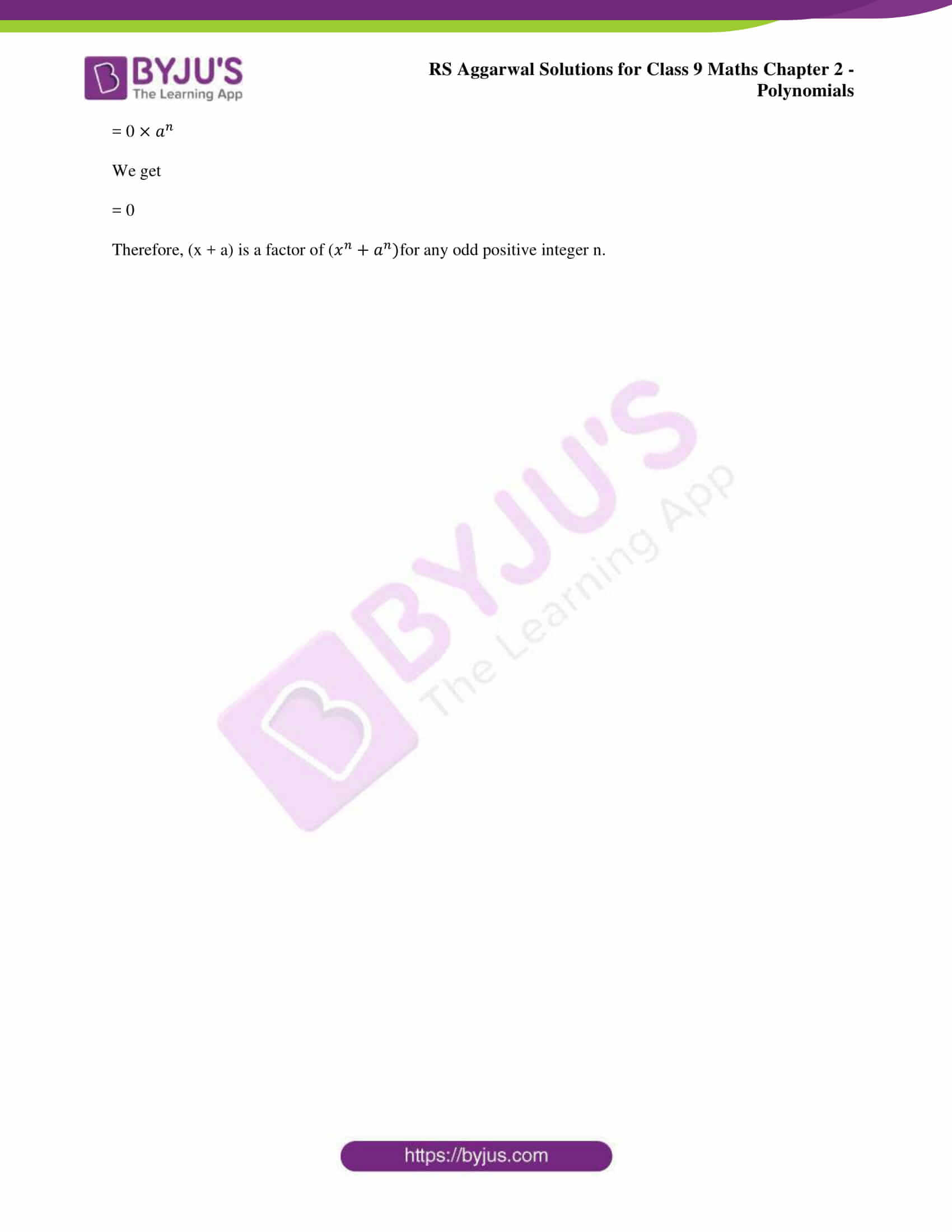### RS Aggarwal Solutions for Class 9 Maths Chapter 2: Polynomials

Chapter 2, Polynomials, has 4 exercises and the RS Aggarwal Solutions has answers in brief to help the students be clear with the concepts.The main topics which are covered in this chapter are:

• Algebraic Expressions
• Terms of an Algebraic Expression
• Polynomials in one variable
• Polynomials of Various degrees
• Number of Terms in a Polynomial
• Value of a Polynomial
• Zeros of a Polynomial
• Division Algorithm in Polynomials
• Remainder Theorem
• Factor Theorem

### RS Aggarwal Solutions Class 9 Maths Chapter 2 – Polynomials

Polynomials is the second chapter in RS Aggarwal textbook where various concepts regarding it are covered. Polynomials are those which contain coefficients with integer exponents, variable and are positive in nature. We make use of polynomial equations in order to explain major areas of Science and Mathematics.

Various other areas where we make use of polynomials are Numerical Analysis, Economics, Chemistry, Physics, Algebraic Geometry, Calculus and Social Science. RS Aggarwal Solutions for Class 9 Chapter 2 Polynomials helps students to revise the topics in an easier way before the exam.